• 决策树前言一、实验目的二、实验内容1、导入数据2、将数据分为训练集与测试集3、采用训练集建立决策树三、实验结果1、Cart算法绘制决策树后记 前言 五一假期跳过了一次实验课，结果这周五上实验课老师竟然直接跳过了...
决策树前言一、实验目的二、实验内容1、导入数据2、将数据分为训练集与测试集3、采用训练集建立决策树三、实验结果1、Cart算法绘制决策树后记
前言
五一假期跳过了一次实验课，结果这周五上实验课老师竟然直接跳过了决策树的实验，虽然说我也不能算是白写了，毕竟算是锻炼自己，不过总还是有点难受的~哼。
恕我懒得再去调整格式了，下面的内容直接是实验报告的原封内容。
一、实验目的
编程实现两种决策树算法，即ID3算法（以信息增益为特征）和Cart算法（以基尼指数为特征），使用Matlab自带函数。
二、实验内容
1、导入数据
即可得到meas和species两个数据，分别是属性和标签。2、将数据分为训练集与测试集
我们使用上一次实验内容中实现的p次k折交叉验证函数来划分训练集与测试集，但是在调用函数之前，我们需要先把属性与标签合并为一个矩阵。
由于只能将同类别数据合并在同一个矩阵中，因此我们需要把species中的标签值按类别修改为1、2、3，得到下面这样的types矩阵：我写了一个函数来完成这个操作，实际上你可以更简单点，直接1:50，50:100，100:150分别为1、2、3即可
function [types]=tag2num(data1)
%将种类名标签，转换为数字，方便与属性合并后传入k折函数
types=[];
data1=categorical(data1);   % 先将cell转换为categorical数组
species=unique(data1);    % 取出类别

for i=1:length(data1)
for j=1:length(species)
if data1(i)==species(j)
types(end+1)=j; % 按照类别给样本标号
end
end
end
types=types';   % 转置

然后，我们就可以将types和meas合并为iris矩阵，并且调用k折交叉验证函数了：
iris=[meas,types];     %把属性与标签合并
[Train_kcross,Test_kcross]=kcrossvalidation(iris);  %k折分组

3、采用训练集建立决策树
由于新版matlab中没有了依据ID3算法构建决策树的函数，因此我们只用Cart算法的决策树函数fitctree实现。
我们从2中通过k折交叉验证得到的Train和Test集合中分别选取一组作为我们建立决策树用的训练集合测试集：
Train_x=Train_kcross(:,1:4,1,1);   % 将第一组训练样本拆开
Train_y=Train_kcross(:,5,1,1);  	% x是属性组，y是标记值
Train_y_tag=num2tag(Train_y);  	% 把标签值改为标签名，方便作图结果
Test_x=Test_kcross(:,1:4,1,1);    % 将第一组测试样本拆开
Test_y=Test_kcross(:,5,1,1);   	% x是属性组，y是标记值
Test_y_tag=num2tag(Test_y);    % 把标签值改为标签名，方便作图结果

其中，num2tag是一个可选操作，我只是为了让画出来的决策树更为直观而已，函数定义如下：
function [y_tag]=num2tag(num_array)
% 该函数的作用是将标记值转回标记名，用以作图
y_tag={};

for i=1:length(num_array)
if num_array(i)==1
y_tag{end+1}='setosa';
end
if num_array(i)==2
y_tag{end+1}='versicolor';
end
if num_array(i)==3
y_tag{end+1}='virginica';
end
end
y_tag=y_tag';

然后将Train_x和Train_y作为构建参数传入fictree函数，构建决策树：
tree=fitctree(Train_x,Train_y_tag,'PredictorNames',{'SepalLength','SepalWidth','PetalLength','PetalWidth'});
view(tree,'Mode','Graph');  %生成决策树并绘制

三、实验结果
1、Cart算法绘制决策树后记
实际上根据其他班同学发给我的实验内容来看，还应该有id3算法完成决策树构建的内容，虽然matlab自带函数不再提供，但是我也参照一些博客写了一系列可以实现该功能的函数。另外还有一个有关剪枝的实验内容，然而matlab的prune方法单纯只是减去某一层的结点，与我们想要的预剪枝、后剪枝效果不太一样，我也没有做，算了，懒得做了诶


展开全文算法 matlab
• 山东大学计算机学院机器学习课程的实验报告，本文是第八章的决策树实验报告
• 实验原理】 决策树(Decision Tree）是在已知各种情况发生概率的基础上，通过...在机器学习中，决策树是一个预测模型，他代表的是对象属性与对象值之间的一种映射关系。Entropy = 系统的凌乱程度，使用算法ID3,...
【实验原理】

决策树(Decision Tree）是在已知各种情况发生概率的基础上，通过构成决策树来求取净现值的期望值大于等于零的概率，评价项目风险，判断其可行性的决策分析方法，是直观运用概率分析的一种图解法。由于这种决策分支画成图形很像一棵树的枝干，故称决策树。在机器学习中，决策树是一个预测模型，他代表的是对象属性与对象值之间的一种映射关系。Entropy = 系统的凌乱程度，使用算法ID3, C4.5和C5.0生成树算法使用熵。这一度量是基于信息学理论中熵的概念。

比如说我们想养一只宠物，但是不知道养哪种宠物比较合适。其实我们可以绘制一个决策树，如下图所示：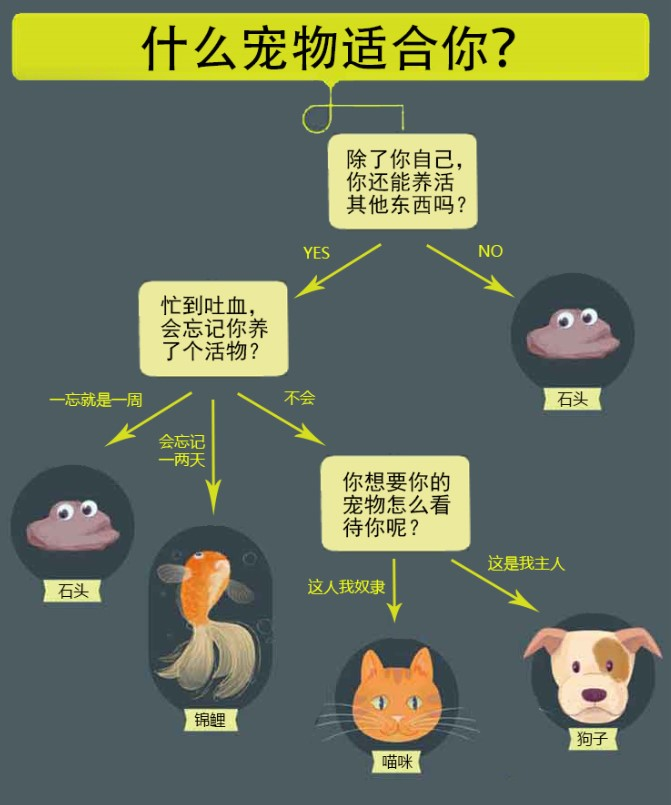1、决策树的算法原理

（1）找到划分数据的特征，作为决策点

（2）利用找到的特征对数据进行划分成n个数据子集。

（3）如果同一个子集中的数据属于同一类型就不再划分，如果不属于同一类型，继续利用特征进行划分。

（4）指导每一个子集的数据属于同一类型停止划分。

2、决策树的优点：计算复杂度不高，输出结果易于理解，对中间值的缺失不敏感，可以处理不相关的特征数据

缺点：可能产生过度匹配的问题

3、那么我们该如何构建一颗决策树呢？在构建一颗决策树的时候我们需要解决的问题有三个：根结点放置哪个条件属性；下面的结点放置哪个属性；什么时候停止树的生长。

为了解决上面三个问题，我们需要引入一些概念。

（1）第一个引入的概念叫信息熵，英文名为 Entropy。在 Tom Mitchell 的书中是这样解释信息熵的：它确定了要编码集合 S 中任意成员（即以均匀的概率随机抽出的一个成员）的分类所需要的最少二进制位数。把它转化成通俗点的语言就是说，信息熵就是“预测随机变量Y的取值”的难度，或者说度量“随机变量Y”的不确定性。

当一个事情非常容易判断的时候，也就是我们以很大的概率认为它会发生或者不会发生，那么它的信息熵就偏向0，当一个事情非常难判断的时候，我们可以认为最难的时候就是这个事件的所有可能性均相等的时候，那么它的信息熵为1。

有很多学者都提出了他们认为的信息熵表达式，我们可以通过下面这个表格看一下目前的一些信息熵的定义。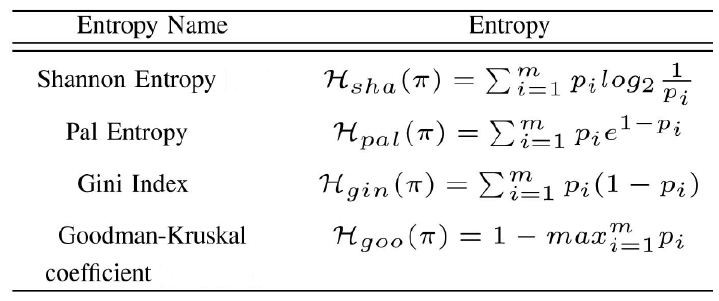虽然有这么多的定义，但我们平时很多情况下用的都是香农信息熵。

（2）有了信息熵的概念之后，我们自然而然就可以得出条件信息熵的概念，条件信息熵就是度量“在随机变量X的前提下，预测随机变量Y”的难度。那么条件信息熵的数学表达式就是：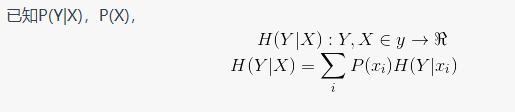（3）有了信息熵和条件信息熵的概念，那我们就自然而然地就可以引出第三个概念，那就是信息增益，信息增益的数学定义是：我们通过看这个数学表达式不难看出信息增益所表达的意思。被减数是信息熵，也就是在没人给我们通风报信的时候判断结果的难度；减数是条件信息熵，也就是当我们知道了一个条件后，判断结果的难度。信息增益这个变量表达的意思就是条件x对判断结果减少了多少难度，即度量X对预测Y的能力的影响。

比方说参加问答，当答题选手无法判断答案的时候会选择三种求助方式，其实求助方式就是一种条件，当选手用过了求助方式后对回答问题的难度的减少量，就是信息增益。如果难度降低很大，那么我们就可以说信息增益很大。

【实验环境】

环境一：直接在百度AI Studio上创建一个项目即可。

环境二：

Ubuntu 16.04

Anaconda 4.3

python 3.6

Pycharm（Community）

【实验内容】

本次实验任务我们使用贷款申请样本数据表，该数据表中每列数据分别代表ID、年龄、高薪、有房、信贷情况、类别，我们根据如下数据生成决策树，使用代码来实现该决策树算法。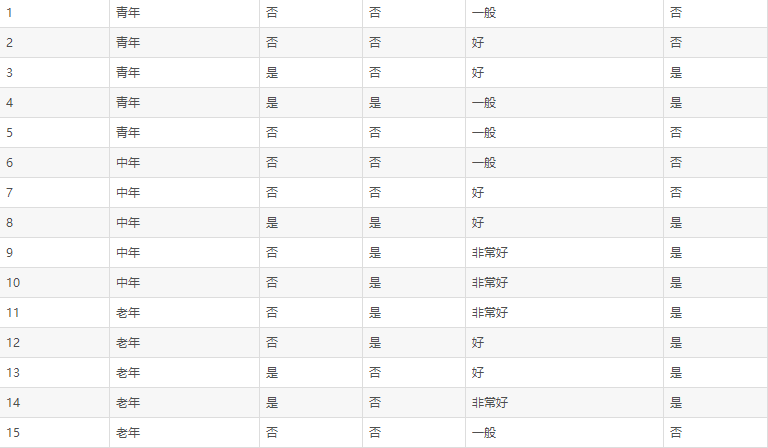【实验步骤】

1.构建决策树如下：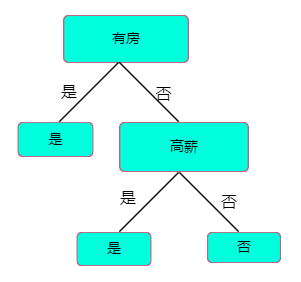该决策树的结构，可以用字典表示为：

{'有自己的房子': {0: {'有工作': {0: 'no', 1: 'yes'}}, 1: 'yes'}}

接下来我们编写python代码来递归构建决策树。



# -*- coding: UTF-8 -*-
from math import log
import operator

#计算数据集的香农商
def calcShannonnt(dataSet):
numEntires = len(dataSet)  #返回数据集的行数
labelCounts = {}   #保存每个标签（Label）出现次数的字典
for featVec in dataSet:  #对每组特征向量进行统计
currentLabel = featVec[-1]  #提取标签Label的信息
if currentLabel not in labelCounts.keys():
#如果标签label没有放入统计次数的字典，则添加进去
labelCounts[currentLabel] = 0
labelCounts[currentLabel] += 1 #Label计数
shannonEnt = 0.0  #香农熵
for key in labelCounts:  #计算香农熵
prob = float(labelCounts[key])/numEntires  #选择标签Label的概率
shannonEnt -= prob * log(prob,2)  #利用计算公式
return shannonEnt
#创建测试数据集
def testDataset():
dataSet = [[0, 0, 0, 0, 'no'],
[0, 0, 0, 1, 'no'],
[0, 1, 0, 1, 'yes'],
[0, 1, 1, 0, 'yes'],
[0, 0, 0, 0, 'no'],
[1, 0, 0, 0, 'no'],
[1, 0, 0, 1, 'no'],
[1, 1, 1, 1, 'yes'],
[1, 0, 1, 2, 'yes'],
[1, 0, 1, 2, 'yes'],
[2, 0, 1, 2, 'yes'],
[2, 0, 1, 1, 'yes'],
[2, 1, 0, 1, 'yes'],
[2, 1, 0, 2, 'yes'],
[2, 0, 0, 0, 'no']]
labels = ['年龄', '高薪', '有房', '信用情况']  #特征标签
return dataSet, labels

#创建函数splitDataset，按照特征划分数据集。
#axis：划分数据集的特征，value：需要返回的特征值
def splitDataset(dataSet, axis, value):
retDataSet = []                                     #创建返回的数据集列表
for featVec in dataSet:                             #遍历数据集
if featVec[axis] == value:
reducedFeatVec = featVec[:axis]             #去掉axis特征
reducedFeatVec.extend(featVec[axis+1:])     #将符合条件的添加到返回的数据集中
retDataSet.append(reducedFeatVec)
return retDataSet
#选择最优特征
def chooseBestFeatureToSplit(dataSet):
numFeatures = len(dataSet) - 1                   #特征数量
baseEntropy = calcShannonent(dataSet)               #计算数据集的香农熵
bestInfoGain = 0.0                                  #信息增益
bestFeature = -1                                    #最优特征的索引值
for i in range(numFeatures):                        #遍历所有特征
#获取dataSet的第i个所有特征
featList = [example[i] for example in dataSet]
uniqueVals = set(featList)                      #创建set集合{},元素不可重复
newEntropy = 0.0                                #经验条件熵
for value in uniqueVals:                        #计算信息增益
subDataSet = splitDataset(dataSet, i, value)        #subDataSet划分后的子集
prob = len(subDataSet) / float(len(dataSet))        #计算子集的概率
newEntropy += prob * calcShannonent(subDataSet)     #根据公式计算经验条件熵
infoGain = baseEntropy - newEntropy                     #信息增益
print("第%d个特征的增益为%.3f" % (i, infoGain))        #打印每个特征的信息增益
if (infoGain > bestInfoGain):                           #计算信息增益
bestInfoGain = infoGain                             #更新信息增益，找到最大的信息增益
bestFeature = i                                     #记录信息增益最大的特征的索引值
return bestFeature

#统计classList中出现次数最多的元素(类标签)。
def createTree(dataSet, labels, featLabels):
classList = [example[-1] for example in dataSet]            #取分类标签(是否放贷:yes or no)
if classList.count(classList) == len(classList):         #如果类别完全相同则停止继续划分
return classList
if len(dataSet) == 1:                                    #遍历完所有特征时返回出现次数最多的类标签
return mostTags(classList)
bestFeat = chooseBestFeatureToSplit(dataSet)                #选择最优特征
bestFeatLabel = labels[bestFeat]                            #最优特征的标签
featLabels.append(bestFeatLabel)
myTree = {bestFeatLabel:{}}                                 #根据最优特征的标签生成树
del(labels[bestFeat])                                       #删除已经使用特征标签
featValues = [example[bestFeat] for example in dataSet]     #得到训练集中所有最优特征的属性值
uniqueVals = set(featValues)                                #去掉重复的属性值
for value in uniqueVals:                                    #遍历特征，创建决策树。
myTree[bestFeatLabel][value] = createTree(splitDataset(dataSet, bestFeat, value), labels, featLabels)
return myTree

def classify(inputTree,featLabels,testVec):
firstStr=next(iter(inputTree))
secondDict=inputTree[firstStr]
featIndex=featLabels.index(firstStr)
for key in secondDict.keys():
if testVec[featIndex]==key:
if type(secondDict[key]).__name__=='dict':
classLabel=classify(secondDict[key],featLabels,testVec)
else:
classLabel=secondDict[key]
return classLabel

if __name__ == '__main__':
dataSet, labels = testDataset()
featLabels = []
myTree = createTree(dataSet, labels, featLabels)
print(myTree)

testVec=[0,1]
result=classify(myTree,featLabels,testVec)
if result=='yes':
print("同意贷款")
if result=='no':
print("拒绝贷款")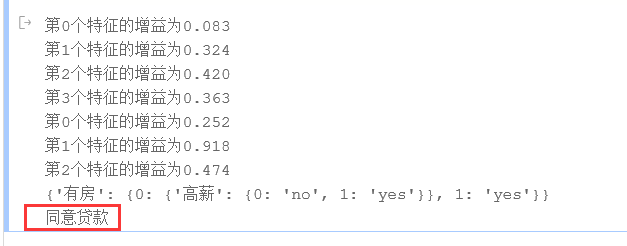展开全文算法 数据挖掘
• 本文是我学习决策树算法的一些总结。 机器学习简介机器学习 (Machine Learning) 是近 20 多年兴起的一门多领域交叉学科，涉及概率论、统计学、逼近论、凸分析、算法复杂度理论等多门学科。简而言之，机器学习是通过...
决策树学习总结

机器学习的应用越来越广泛，特别是在数据分析领域。本文是我学习决策树算法的一些总结。

机器学习简介

机器学习 (Machine Learning) 是近 20 多年兴起的一门多领域交叉学科，涉及概率论、统计学、逼近论、凸分析、算法复杂度理论等多门学科。简而言之，机器学习是通过学习老知识（训练样本），得出自己的认知（模型），去预测未知的结果。

学习方式 监督式学习 从给定的训练数据集中学习出一个函数，当新的数据到来时，可以根据此函数预测结果。训练数据集中的目标由人标注的。常见的算法有回归分析和统计分类
非监督式学习 与监督式学习相比，训练集没有人为标注的结果，常见的算法有聚类
半监督式学习 训练集部分被标识，部分没有被标识。常见的算法有SVM
强化学习 输入数据作为模型的反馈，模型对此作出调整。常见的算法有时间差学习
机器学习算法分类 决策树算法 根据数据属性，采用树状结构建立决策模型。常用来解决分类和回归问题。
常见算法：CART(Classification And Regression Tree)，ID3，C4.5，随机森林等
回归算法 对连续值预测，如逻辑回归LR等
分类算法 对离散值预测，事前已经知道分类，如k-近邻算法
聚类算法 对离散值预测，事前对分类未知，如k-means算法
神经网络 模拟生物神经网络，可以用来解决分类和回归问题
感知器神经网络(Perceptron Neural Network) ，反向传递(Back Propagation)和深度学习（DL）
集成算法 集成几种学习模型进行学习，将最终预测结果进行汇总
决策树算法

初识决策树
决策树算法是借助于树的分支结构实现分类。以相亲约会决策为例，下图是建立好的决策树模型，数据的属性有4个：年龄、长相、收入、是否公务员，根据此模型，可以得到最终是见或者不见。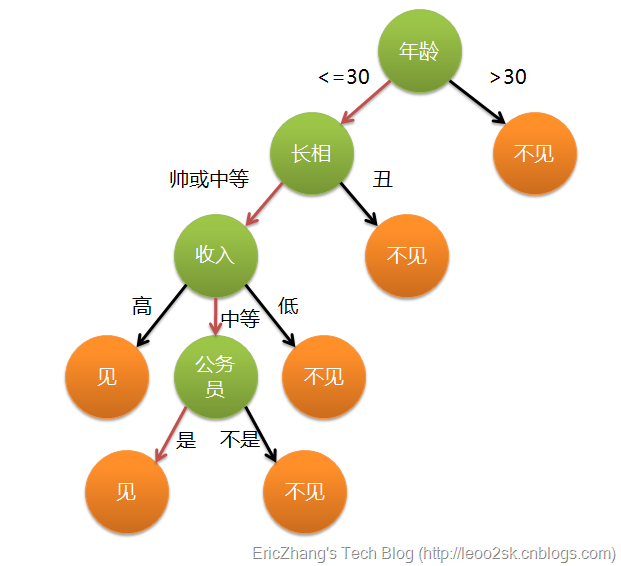这样，我们对决策树有个初步认识：

叶子节点：存放决策结果
非叶子节点：特征属性，及其对应输出，按照输出选择分支
决策过程：从根节点出发，根据数据的各个属性，计算结果，选择对应的输出分支，直到到达叶子节点，得到结果
构建决策树
通过上述例子，构建过程的关键步骤是选择分裂属性，即年龄、长相、收入、公务员这4个属性的选择先后次序。分裂属性是在某个节点处按照某一特征属性的不同划分构造不同的分支，其目标是让各个分裂子集尽可能的“纯”，即每个子集尽量都属于同一分类项。分裂属性分3种情况：

属性是离散值且不要求生成二叉树 属性的每个值作为一个分支
属性是离散值且要求生成二叉树 按照“属于”和“不属于”分成2个分支
属性是连续值 确定一个分裂点split_point，按照>split_point和<=split_point生成2个分支注意，决策树使用自顶向下递归分治法，并采用不回溯的贪心策略。分裂属性的选择算法很多，这里介绍3种常用的算法：信息增益（Information gain）、增益比率（gain ratio）、基尼指数（Gini index）
信息增益（Information Gain）
基于香浓的信息论，信息熵表示不确定度，均匀分布时，不确定度最大，此时熵就最大。当选择某个特征对数据集进行分类时，数据集分类后的信息熵会比分类前的小，其差值即为信息增益。信息增益可以衡量某个特征对分类结果的影响大小，越大越好。 典型算法：ID3
数据集D中，有m个类别，$$p_i$$表示D中属于类别i的概率，此数据集的信息熵定义为：Info(D)=−∑_i=1mp_ilog_2(p_i)<!--//--><![CDATA[//><!--
Info(D)=-\sum\_{i=1}^{m}p\_i\log\_2(p\_i)
//--><!]]>
以属性R作为分裂属性，R有k个不同的取值，将数据D划分成k组，按R分裂后的数据集的信息熵为：Info_R(D)=∑_j=1k|D_j||D|×Info(D_j)<!--//--><![CDATA[//><!--
Info\_R(D)=\sum\_{j=1}^{k}\frac{|D\_j|}{|D|}\times Info(D\_j)
//--><!]]>
信息增益，即为划分前后，信息熵之差：Gain(R)=Info(D)−InfoR(D)<!--//--><![CDATA[//><!--
Gain(R)=Info(D)-Info_R(D)
//--><!]]>
在每层分裂时，选择使得Gain(R)最大的属性作为分裂属性
缺点：此公式偏向数据量多的属性，如果样本分布不均，则会导致过拟合。假如上述例子中包括人名属性，每个人名均不同，显然以此属性作为划分，信息增益最高，但是，很明显，以此属性作为划分毫无意义
信息增益比率（Gain Ratio）
针对上述方法问题，此方法引入分裂信息SplitInfo_R(D)=−∑_j=1k|D_j|D×log_2(|D_j|D))<!--//--><![CDATA[//><!--
SplitInfo\_R(D)=-\sum\_{j=1}^{k}\frac{|D\_j|}{D}\times \log\_{2}(\frac{|D\_j|}{D}))
//--><!]]>典型算法：C4.5
信息增益比率定义为：GainRatio(R)=Gain(R)SplitInfo_R(D)<!--//--><![CDATA[//><!--
GainRatio(R)=\frac{Gain(R)}{SplitInfo\_R(D)}
//--><!]]>
缺点：$$SplitInfo_R(D)$$可能取值为0，此时无意义；当期趋于0时，GainRatio也不可信，改进措施是在分母加一个平滑，这里加所有分裂信息的平均值$$GainRatio(R)=\frac{Gain(R)}{\overline{SplitInfo(D)}+SplitInfo_R(D)}$$
基尼指数（Gini index）
另外一种数据不纯度的度量方法，定义为：Gini(D)=1−∑_i=1mp_i2<!--//--><![CDATA[//><!--
Gini(D)=1-\sum\_{i=1}^{m}{p\_{i}}^{2}
//--><!]]>
其中，m为数据集D中类别的个数，$$p_i$$表示D中属于类别i的概率，如果所有记录都属于同一个类中，则P1=1，Gini(D)=0。 典型算法：CART
以属性R作为分裂属性，R有k个不同的取值，将数据D划分成k组，按R分裂后的数据集的基尼指数为：Gini_R(D)=∑_i=1k|D_i||D|Gini(D_i)<!--//--><![CDATA[//><!--
Gini\_R(D)=\sum\_{i=1}^{k}\frac{|D\_i|}{|D|}Gini(D\_i)
//--><!]]>
计算划分前后基尼指数之差△Gini(R)=Gini(D)−Gini_R(D)<!--//--><![CDATA[//><!--
\bigtriangleup Gini(R)=Gini(D)-Gini\_R(D)
//--><!]]>计算Gini(R)增量最大的属性作为最佳分裂属性。
spark中实现

具体代码参考spark源码包下的org.apache.spark.examples.mllib.DecisionTreeClassificationExample，DecisionTree.trainClassifier的实现步骤，核心代码在RandomForest.run()方法

根据输入数据，构建RDD[LabeledPoint]，保存label和features
根据数据，构建metaData，包括feature个数、样本数据条数、分类个数等 val metadata =
DecisionTreeMetadata.buildMetadata(retaggedInput, strategy, numTrees, featureSubsetStrategy)
计算每个feature的可能划分点split1、split2。。。split(n-1)划分成n个bin val splits = findSplits(retaggedInput, metadata, seed)
连续值：取样数据，根据不同值个数和步长进行划分
离散： unorder：特征种类为binsNum（分类个数不大，且为多分类）
order：回归、二分类、多分类且数量很大，都使用二分类
根据feature的split，计算每条数据在每个feature下所在的bin，生成新的RDD[TreePoint(Label, featuresBin[])] val treeInput = TreePoint.convertToTreeRDD(retaggedInput, splits, metadata)
bagging取样（示例中，不需要取样，因为只生成一个tree） val baggedInput = BaggedPoint
.convertToBaggedRDD(treeInput, strategy.subsamplingRate, numTrees, withReplacement, seed)
.persist(StorageLevel.MEMORY_AND_DISK)
新建树根root，放到queue中，循环直到队列为空 选择多个训练树节点，同时进行训练，根据指定的maxMemoryUsage进行个数计算 val (nodesForGroup, treeToNodeToIndexInfo) =
RandomForest.selectNodesToSplit(nodeQueue, maxMemoryUsage, metadata, rng)
按照Gini系数，找到当前树节点中，最佳的feature分裂点和最佳bin分割点 RandomForest.findBestSplits(baggedInput, metadata, topNodes, nodesForGroup,
treeToNodeToIndexInfo, splits, nodeQueue, timer, nodeIdCache)
遍历数据，mapPartition，每个树节点LearnNode维护一个DTStatsAggregator（存放，每个feature的每个bin的数据个数）
进行DTStatsAggregator的聚合merge
按照Gini系数，找到最佳的feature下的最佳split
参数设置

可以通过设置一些参数，调整算法或调优，详细参考spark官网介绍

算法设置 algo：决策树类型，分类或回归，Classification or Regression
numClasses： 分类问题设置分类个数
categoricalFeaturesInfo：指定哪些feature是离散值，并指定离散值的个数
停止条件设置 maxDepth：树最大深度
minInstancesPerNode：每个树节点最小的训练数据个数，如果小于此值，则不进行分裂
minInfoGain：最小信息增益，决定当前树节点是否进行分裂
调优参数 maxBins：用于连续特征值的分散化，最多划分类个数
maxMemoryInMB：增大，可以提高同时训练的树节点的个数，从而减少训练时间，但是，每次训练会增大数据的传输量
subsamplingRate：训练森林时使用，每次采样的比例
impurity：纯度度量函数，必须和算法algo相匹配
参考文档

决策树算法介绍及应用
数据挖掘系列（6）决策树分类算法
wikipedia_decisiontree

展开全文spark
• 机器学习决策树的随机森林 机器学习 (Machine Learning) Machine learning is an application of artificial intelligence that provides systems the ability to automatically learn and improve from ...
机器学习中决策树的随机森林 机器学习 (Machine Learning)
Machine learning is an application of artificial intelligence that provides systems the ability to automatically learn and improve from experience without being explicitly programmed. The 3 main categories of machine learning are supervised learning, unsupervised learning, and reinforcement learning. In this post, we shall focus on supervised learning for classification problems. 中号 achine学习是人工智能，它提供系统能够自动学习并从经验中提高，而不明确地编程能力的应用。 机器学习的3个主要类别是监督学习，无监督学习和强化学习。 在这篇文章中，我们将专注于分类问题的监督学习。
Supervised learning learns from past data and applies the learning to present data to predict future events. In the context of classification problems, the input data is labeled or tagged as the right answer to enable accurate predictions. 监督学习从过去的数据中学习，并将学习应用于当前的数据以预测未来的事件。 在分类问题的上下文中，将输入数据标记或标记为正确答案，以实现准确的预测。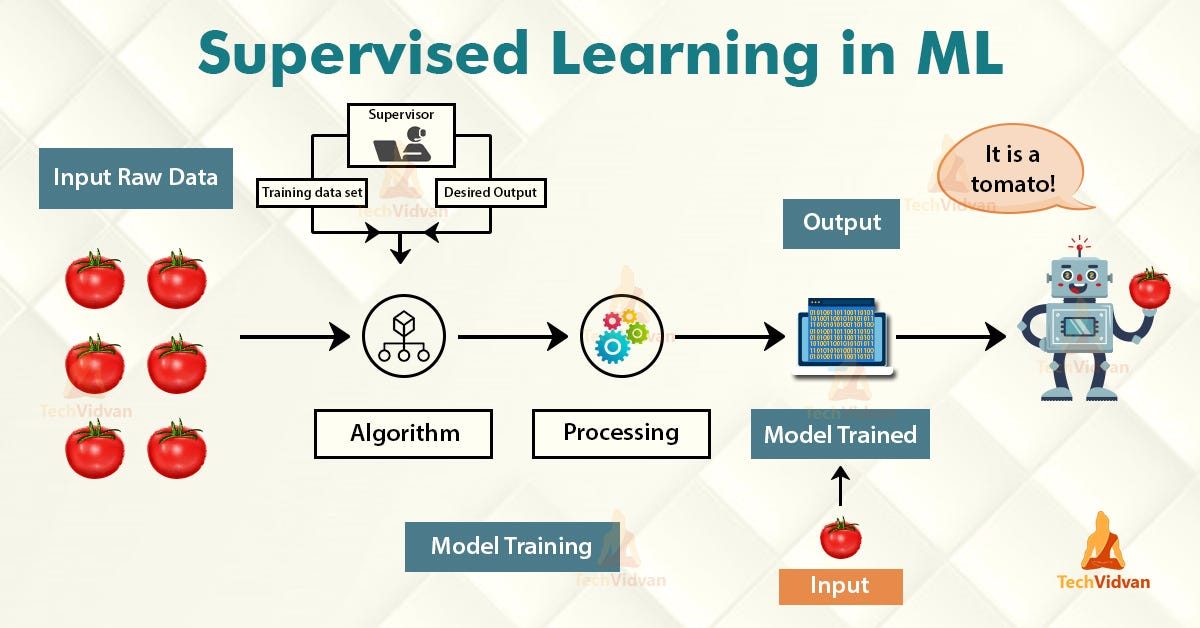TechvidvanTechvidvan Tree-based learning algorithms are one of the most commonly used supervised learning methods. They empower predictive models with high accuracy, stability, ease of interpretation, and are adaptable at solving any classification or regression problem. 基于树的学习算法是最常用的监督学习方法之一。 它们使预测模型具有较高的准确性，稳定性，易解释性，并且适用于解决任何分类或回归问题。
Decision Tree predicts the values of responses by learning decision rules derived from features. In a tree structure for classification, the root node represents the entire population, while decision nodes represent the particular point where the decision tree decides on which specific feature to split on. The purity for each feature will be assessed before and after the split. The decision tree will then decide to split on a specific feature that produces the purest leaf nodes (ie. terminal nodes at each branch). 决策树通过学习从要素派生的决策规则来预测响应的值。 在用于分类的树结构中 ， 根节点代表整个种群，而决策节点则代表决策树确定要分割的特定特征的特定点。 在拆分之前和之后，将评估每个功能部件的纯度。 然后，决策树将决定拆分产生一个最纯叶节点 (即每个分支的终端节点)的特定功能。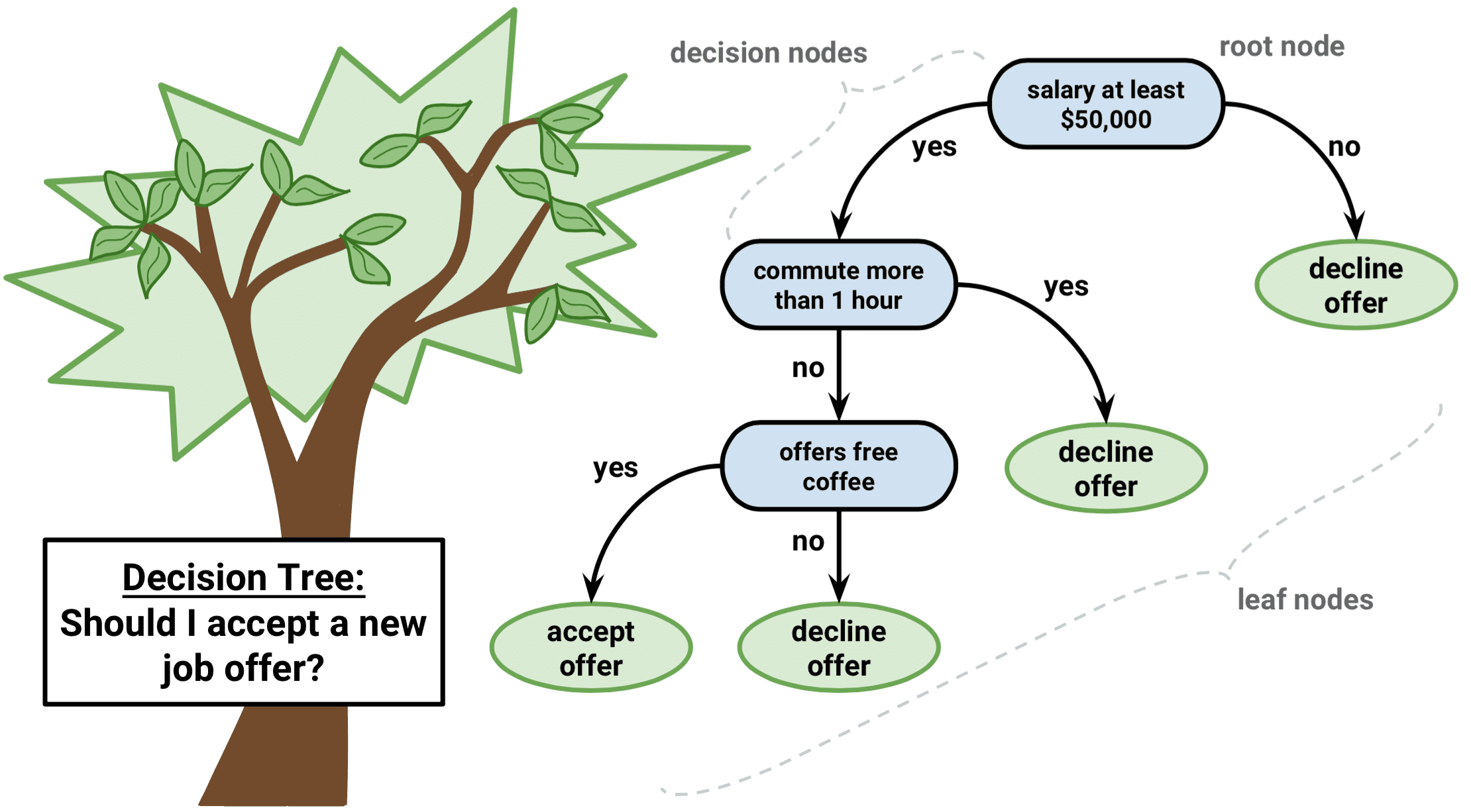Datacamp)Datacamp ) A significant advantage of a decision tree is that it forces the consideration of all possible outcomes of a decision and traces each path to a conclusion. It creates a comprehensive analysis of the consequences along each branch and identifies decision nodes that need further analysis. 决策树的显着优势在于，它可以强制考虑决策的所有可能结果，并跟踪得出结论的每条路径。 它对每个分支的后果进行全面分析，并确定需要进一步分析的决策节点。
However, a decision tree has its own limitations. The reproducibility of the decision tree model is highly sensitive, as a small change in the data can result in a large change in the tree structure. Space and time complexity of the decision tree model is relatively higher, leading to longer model training time. A single decision tree is often a weak learner, hence a bunch of decision tree (known as random forest) is required for better prediction. 但是， 决策树有其自身的局限性 。 决策树模型的可重复性非常敏感，因为数据的微小变化会导致树形结构的巨大变化。 决策树模型的时空复杂度相对较高，导致模型训练时间更长。 单个决策树通常学习能力较弱，因此需要一堆决策树(称为随机森林)才能更好地进行预测。
The random forest is a more powerful model that takes the idea of a single decision tree and creates an ensemble model out of hundreds or thousands of trees to reduce the variance. Thus giving the advantage of obtaining a more accurate and stable prediction. 随机森林是一个功能更强大的模型，它采用单个决策树的概念，并从数百或数千棵树中创建一个集成模型以减少差异。 因此具有获得更准确和稳定的预测的优势 。
Each tree is trained on a random set of observations, and for each split of a node, only a random subset of the features is used for making a split. When making predictions, the random forest does not suffer from overfitting as it averages the predictions for each of the individual decision trees, for each data point, in order to arrive at a final classification. 每棵树都在一组随机的观测值上训练，并且对于节点的每个拆分，仅使用特征的随机子集进行拆分。 在进行预测时，随机森林不会遭受过度拟合的影响，因为它会针对每个数据点对每个单独决策树的预测取平均，以得出最终分类。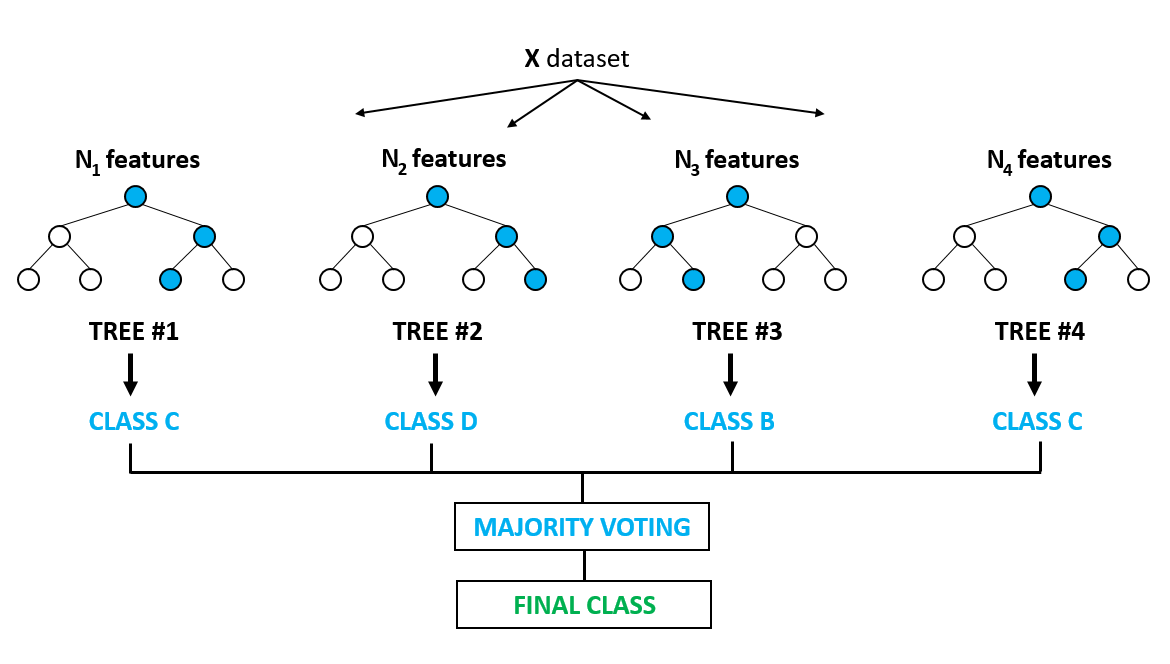Abilash R)Abilash R ) We shall approach a classification problem and explore the basics of how decision trees work, how individual decisions trees are combined to form a random forest, how to fine-tune the hyper-parameters to optimize random forest, and ultimately discover the strengths of using random forests. 我们将研究分类问题，并探索决策树如何工作，如何将单个决策树组合以形成随机森林，如何微调超参数以优化随机森林的基础知识，并最终发现使用随机森林的优势。森林。
问题陈述：预测一个人每年的收入是否超过50,000美元。 (Problem Statement: To predict whether a person makes more than U$50,000 per year.) 让我们开始编码！ (Let’s start coding!) import pandas as pd import numpy as np from sklearn.preprocessing import LabelEncoder from sklearn.feature_selection import SelectKBest from sklearn.feature_selection import chi2 from sklearn.utils import resample from sklearn.model_selection import train_test_split from sklearn import preprocessing from sklearn.tree import DecisionTreeClassifier from sklearn.tree import plot_tree from sklearn.ensemble import RandomForestClassifier from sklearn.model_selection import GridSearchCV from sklearn import metrics from sklearn.metrics import accuracy_score, precision_score, recall_score, f1_score from sklearn.metrics import classification_report, precision_recall_curve, roc_curve, roc_auc_score import scikitplot as skplt import matplotlib.pyplot as plt import seaborn as sns %matplotlib inline 资料准备 (Data Preparation) Load the Census Income Dataset from the URL and display the top 5 rows to inspect the data. 从URL加载人口普查收入数据集 ，并显示前5行以检查数据。 # Add header=None as the first row of the file contains the names of the columns. # Add engine='python' to avoid parser warning raised for reading a file that doesn’t use the default ‘c’ parser. income_data = pd.read_csv('https://archive.ics.uci.edu/ml/machine-learning-databases/adult/adult.data', header=None, delimiter=', ', engine='python') income_data.head()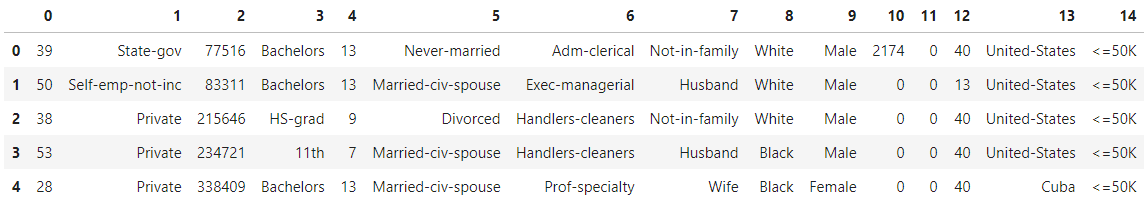# Add headers to dataset headers = ['age','workclass','fnlwgt','education','education_num','marital_status','occupation','relationship', 'race','sex','capital_gain','capital_loss','hours_per_week','native_country','income'] income_data.columns = headers income_data.head()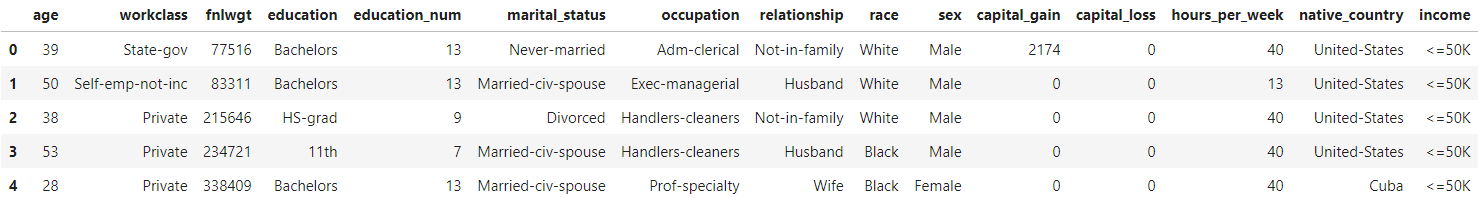数据清理 (Data Cleaning) # Check for empty cells and if data types are correct for the respective columns income_data.info()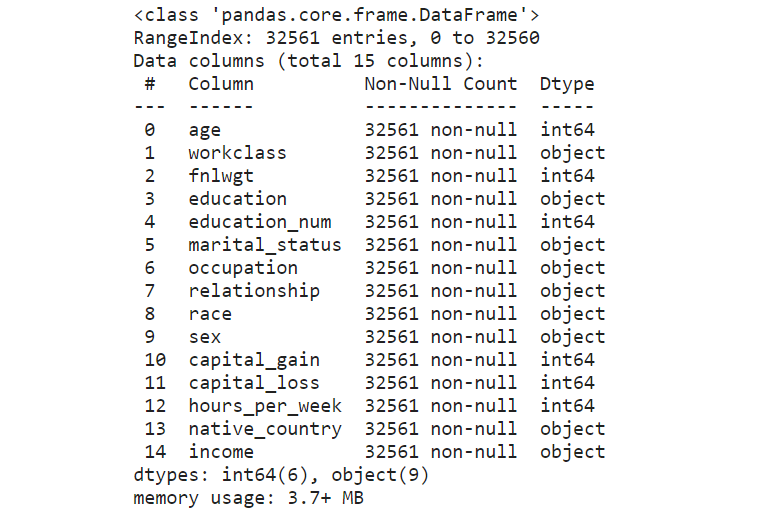Box plots are useful as they show outliers for integer data types within a data set. An outlier is an observation that is numerically distant from the rest of the data. When reviewing a box plot, an outlier is defined as a data point that is located outside the whiskers of the box plot. 箱形图很有用，因为它们显示了数据集中整数数据类型的离群值。 离群值是在数值上与其余数据相距遥远的观测值 。 查看箱形图时，离群值定义为位于箱形图晶须之外的数据点。 # Use a boxplot to detect any outliers income_data.boxplot(figsize=(30,6), fontsize=20);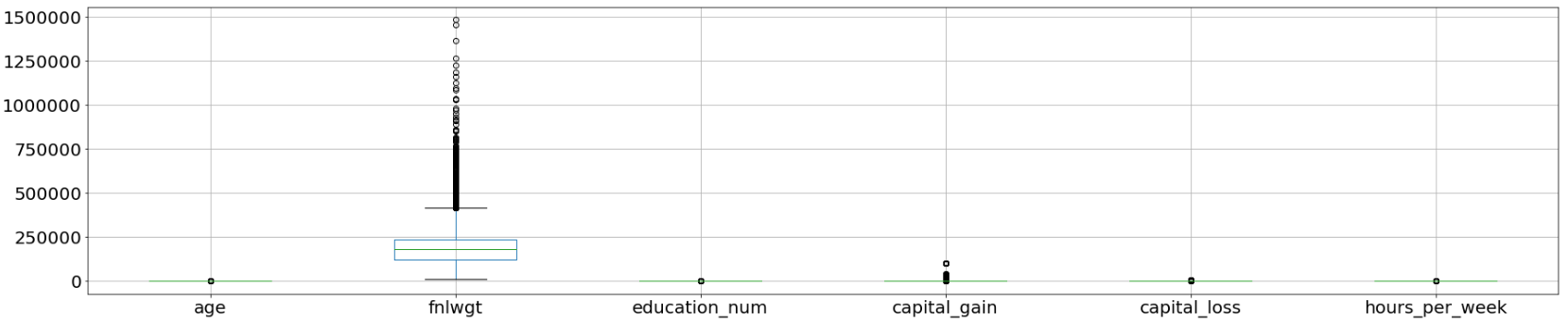As extracted from the attributes listing in the Census Income Data Set, the feature “fnlwgt” refers to the final weight. It states the number of people the census believes the entry represents. Therefore, this outlier would not be relevant to our analysis and we would proceed to drop this column. 从“ 人口普查收入数据集 ”中的属性列表中提取的功能“ fnlwgt”是指最终权重。 它指出了人口普查相信该条目所代表的人数。 因此，此异常值与我们的分析无关，我们将继续删除此列。 clean_df = income_data.drop(['fnlwgt'], axis=1) clean_df.info()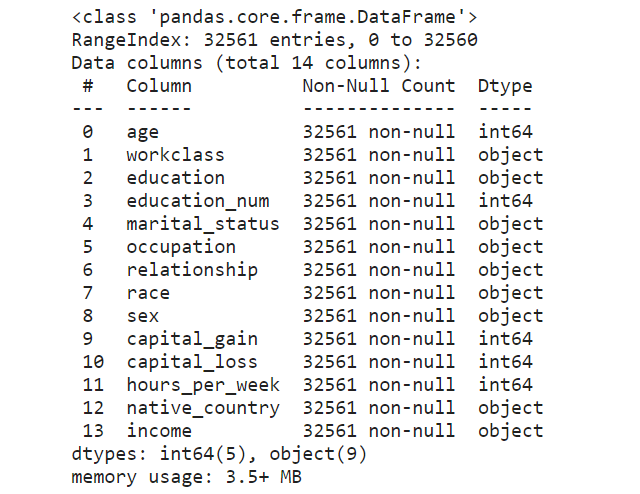# Select duplicate rows except first occurrence based on all columns dup_rows = clean_df[clean_df.duplicated()] dup_rows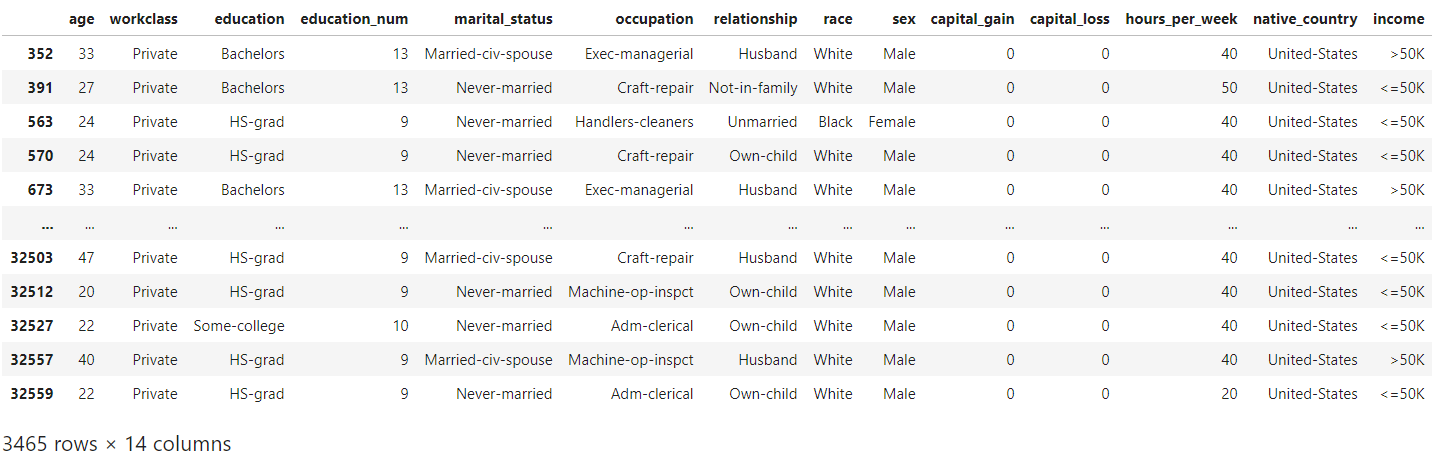An example of duplicates can be seen in the above rows with the entries “Private” under the “workclass” column. These duplicate rows correspond to samples for different surveyed individuals instead of genuine duplicate rows. As such, we would not remove any duplicated rows to preserve the data for further analysis. 可以在上面的行中看到重复的示例，在“工作类别”列下有条目“私人”。 这些重复的行对应于不同调查对象的样本，而不是真正的重复行。 因此，我们不会删除任何重复的行来保留数据以供进一步分析。 标签编码 (Label Encoding) Categorical features are encoded into numerical values using label encoding, to convert each class under the specified feature to a numerical value. 使用标签编码将分类要素编码为数值，以将指定要素下的每个类别转换为数值。 # Categorical boolean mask categorical_feature_mask = clean_df.dtypes==object # Filter categorical columns using mask and turn it into a list categorical_cols = clean_df.columns[categorical_feature_mask].tolist() # Instantiate labelencoder object le = LabelEncoder() # Apply label encoder on categorical feature columns clean_df[categorical_cols] = clean_df[categorical_cols].apply(lambda col: le.fit_transform(col)) clean_df[categorical_cols].head(5)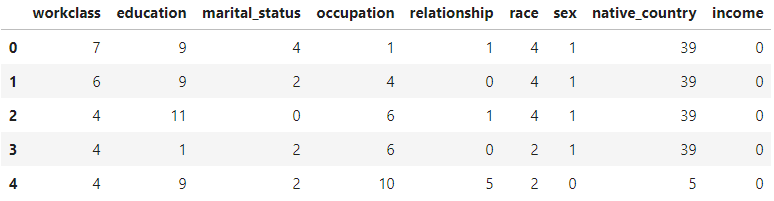X = clean_df.iloc[:,0:13] # independent columns - features y = clean_df.iloc[:,-1] # target column - income # Distribution of target variable print(clean_df["income"].value_counts())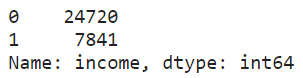print(clean_df["income"].value_counts(normalize=True)) # 0 for label: <= U$50K
# 1 for label: > U$50K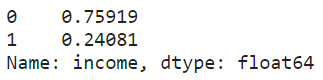An imbalanced dataset was observed from the above-normalized distribution. 从上述归一化分布中观察到不平衡的数据集。 实验设计 (Design of Experiment) It would be interesting to see how different factors can affect the performance of each classifier. Let’s consider the following 3 factors: 看看不同的因素如何影响每个分类器的性能会很有趣。 让我们考虑以下三个因素：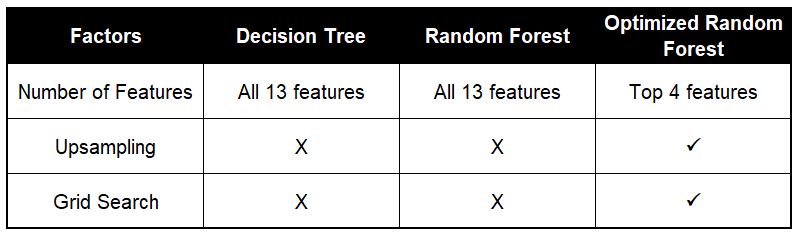Number of features: When deciding on the number of features to use for a particular dataset, The Elements of Statistical Learning (section 15.3) states that: 特征数量：在确定用于特定数据集的特征数量时， 《统计学习的要素》 (第15.3节)指出： Typically, for a classification problem with p features, √p features are used in each split. 通常，对于具有p个特征的分类问题，在每个拆分中使用√p个特征。 Thus, we would perform feature selection to choose the top 4 features for the modeling of the optimized random forest. With the ideal number of features, it would help to prevent overfitting and improve model interpretability. 因此，我们将执行特征选择，以选择用于优化随机森林建模的前4个特征。 具有理想数量的功能，将有助于防止过度拟合并提高模型的可解释性。 Upsampling: An imbalanced dataset would lead to a biased model after training. For this particular dataset, we see a distribution of 76% representing the majority class (ie. income <=U$50K) and the remaining 24% representing the minority class (ie. income >U$50K). 上采样：训练后，不平衡的数据集会导致模型产生偏差。 对于这个特定的数据集，我们看到76％的分布代表多数阶层(即，收入<= U$ 50K)，其余24％的分布代表少数群体(即，收入> U $50K)。 Upon training of the models, we will have the decision tree and random forest achieving a high classification accuracy belonging to the majority class. To overcome this, we would perform an upsampling of the minority class (ie. income >U$50K) to create a balanced dataset for the optimized random forest model. 训练模型后，我们将拥有属于多数类别的，具有较高分类精度的决策树和随机森林。 为了克服这个问题，我们将对少数群体(即收入> 5万美元)进行上采样，以为优化的随机森林模型创建一个平衡的数据集。
Grid search: In order to maximize the performance of the random forest, we can perform a grid search for the best hyperparameters and optimize the random forest model. 网格搜索：为了最大化随机森林的性能，我们可以对最佳超参数执行网格搜索并优化随机森林模型。  资料建模 (Data Modelling)
An initial loading and splitting of the dataset were performed to train and test the decision tree and random forest models, before optimizing the random forest. 在优化随机森林之前，先对数据集进行初始加载和拆分，以训练和测试决策树和随机森林模型。
X_train_bopt, X_test_bopt, y_train_bopt, y_test_bopt = train_test_split(X, y,
test_size = 0.3,
random_state = 1)Standardization of datasets is a common requirement for many machine learning estimators implemented in scikit-learn. The dataset might behave badly if the individual features do not more or less look like standard normally distributed data, ie. Gaussian with zero mean and unit variance. 标准化   对于scikit-learn中实现的许多机器学习估计器，数据集的数量是一个普遍的要求。 如果各个要素看起来或多或少不像标准正态分布数据(即)，则数据集的行为可能会很差。 具有零均值和单位方差的高斯。
# Perform pre-processing to scale numeric features
scale = preprocessing.StandardScaler()
X_train_bopt = scale.fit_transform(X_train_bopt)

# Test features are scaled using the scaler computed for the training features
X_test_bopt = scale.transform(X_test_bopt) 模型1：决策树 (Model 1: Decision Tree)
# Create decision tree classifier
tree = DecisionTreeClassifier(random_state=1)

# Fit training data and training labels to decision tree
tree.fit(X_train_bopt, y_train_bopt)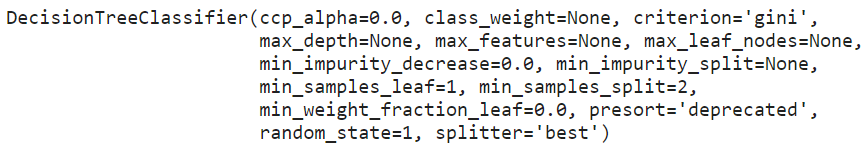print(f'Decision Tree has {tree.tree_.node_count} nodes with a maximum depth of {tree.tree_.max_depth}.')

print(f'Model Accuracy for train data: {tree.score(X_train_bopt, y_train_bopt)}')
print(f'Model Accuracy for test data: {tree.score(X_test_bopt, y_test_bopt)}')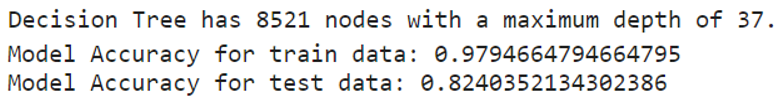As there was no limit on the depth, the decision tree model was able to classify every training point perfectly to a large extent. 由于深度没有限制，因此决策树模型能够在很大程度上对每个训练点进行完美分类。
决策树的可视化 (Visualization of the Decision Tree)
By visualizing the decision tree, it will show each node in the tree which we can use to make new predictions. As the tree is relatively large, the decision tree is plotted below, with a maximum depth of 3. 通过可视化决策树，它将显示树中的每个节点，我们可以使用它们进行新的预测。 由于树比较大，下面绘制了决策树，最大深度为3。
# Create and fit decision tree with maximum depth 3
tree = DecisionTreeClassifier(max_depth=3, random_state=1)
tree.fit(X_train_bopt, y_train_bopt)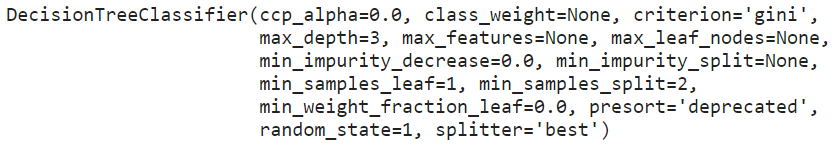# Plot the decision tree
plt.figure(figsize=(25,10))
decision_tree_plot = plot_tree(tree, feature_names=X.columns,
class_names=['<=50K','>50K'],
filled=True, rounded=True, fontsize=14)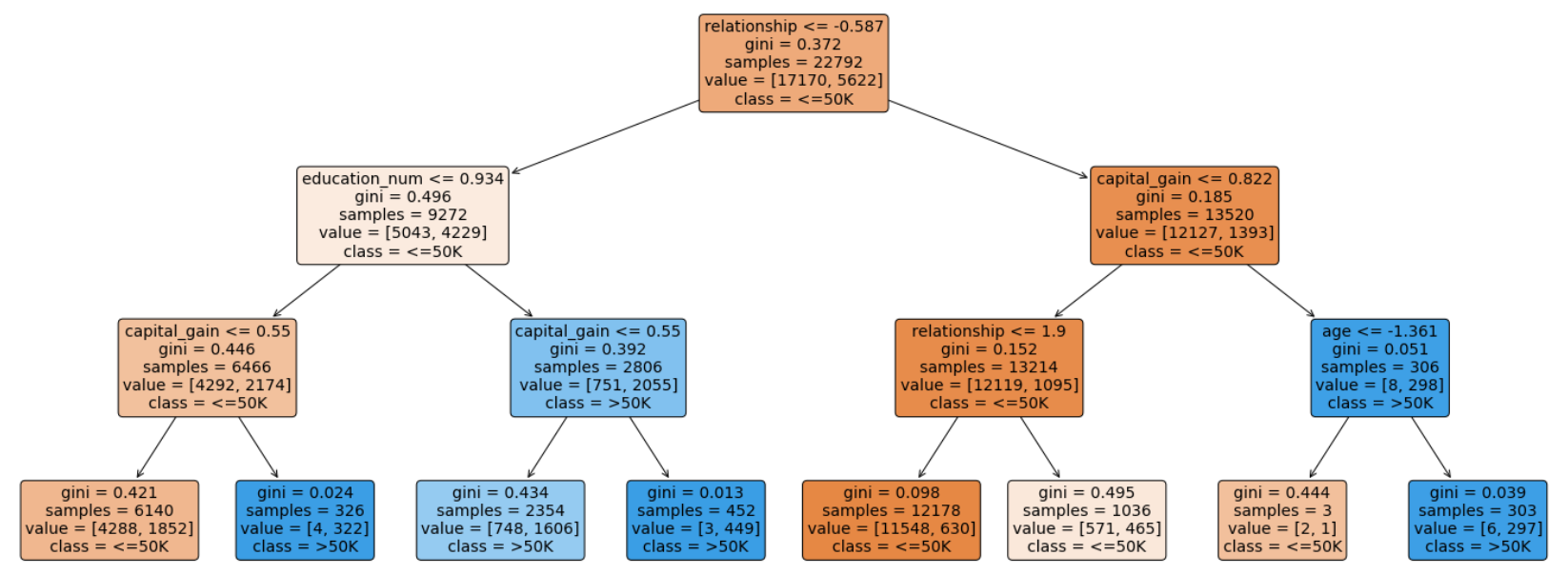对于每个节点(叶节点除外)，五行表示： (For each of the nodes (except the leaf nodes), the five rows represent:)
question asked about the data based on a feature: This determines the way we traverse down the tree for a new data point. question asked about the data based on a feature ：这确定了我们遍历树以获取新数据点的方式。 gini: The gini impurity of the node represents the probability that a randomly selected sample from a node will be incorrectly classified according to the distribution of samples in the node. The average (weighted by samples) gini impurity decreases with each level of the tree. gini ：节点的gini杂质表示从节点中随机选择的样本将根据节点中样本的分布进行错误分类的概率。 树木的每个水平均会降低吉尼杂质的平均值(按样品加权)。 samples: The number of training observations in the node. samples ：节点中训练观测的数量。 value: The number of samples in the respective classes. value ：各个类别中的样本数。 class: The class predicted for all the points in the node if the tree ended at this depth. class ：如果树在此深度处结束，则为节点中所有点预测的类。 The leaf nodes are where the tree makes a prediction. The different colors correspond to the respective classes, with shades ranging from light to dark depending on the gini impurity. 叶子节点是树进行预测的地方。 不同的颜色对应于各个类别，取决于基尼杂质，阴影的范围从浅到深。
修剪决策树 (Pruning the Decision Tree)
Limiting the maximum depth of the decision tree can enable the tree to generalize better to testing data. Although this will lead to reduced accuracy on the training data, it can improve performance on the testing data and provide an objective performance evaluation. 限制决策树的最大深度可以使决策树更好地推广到测试数据。 尽管这将导致训练数据的准确性降低，但可以提高测试数据的性能并提供客观的性能评估。
# Create for loop to prune tree
scores = []

for i in range(1, 31):
tree = DecisionTreeClassifier(random_state=1, max_depth=i)
tree.fit(X_train_bopt, y_train_bopt)
score = tree.score(X_test_bopt, y_test_bopt)
scores.append(tree.score(X_test_bopt, y_test_bopt))

# Plot graph to see how individual accuracy scores changes with tree depth
sns.set_context('talk')
sns.set_palette('dark')
sns.set_style('ticks')

plt.plot(range(1, 31), scores)
plt.xlabel("Depth of Tree")
plt.ylabel("Scores")
plt.title("Decision Tree Classifier Accuracy")
plt.show()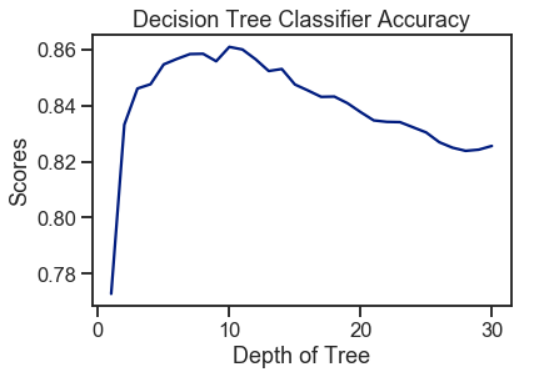Using the decision tree, a peak of 86% accuracy was achieved with an optimal tree depth of 10. As the depth of the tree increases, the accuracy score decreases gradually. Hence, a deeper tree depth does not reflect a higher accuracy for prediction. 使用决策树时，最佳树深度为10时，达到了86％的精度峰值。随着树的深度增加，精度得分逐渐降低。 因此，更深的树深度不能反映更高的预测精度。
模型2：随机森​​林 (Model 2: Random Forest)
包外错误评估 (Out-of-Bag Error Evaluation)
The Random Forest Classifier is trained using bootstrap aggregation, where each new tree is fitted from a bootstrap sample of the training observations. The out-of-bag error is the average error for each training observation calculated using predictions from the trees that do not contain the training observation in their respective bootstrap sample. This allows the Random Forest Classifier to be fitted and validated whilst being trained. 使用引导聚合对随机森林分类器进行训练，其中从训练观测值的引导样本中拟合出每棵新树。 袋外误差是每个训练观测值的平均误差，这些误差是使用来自在其各自的引导样本中不包含训练观测值的树的预测所计算出的。 这允许在训练过程中对随机森林分类器进行拟合和验证。
The random forest model was fitted with a range of tree numbers and evaluated on the out-of-bag error for each of the tree’s numbers used. 随机森林模型配有一系列树木编号，并针对所使用的每个树木编号评估了袋外误差。
# Initialise the random forest estimator
# Set 'warm_start=true' so that more trees are added to the existing model each iteration
RF = RandomForestClassifier(oob_score=True, random_state=1,
warm_start=True, n_jobs=-1)

oob_list = list()

# Iterate through all of the possibilities for the number of trees
for n_trees in [15, 20, 30, 40, 50, 100, 150, 200, 300, 400]:
RF.set_params(n_estimators=n_trees)  # Set number of trees
RF.fit(X_train_bopt, y_train_bopt)
oob_error = 1 - RF.oob_score_ # Obtain the oob error
oob_list.append(pd.Series({'n_trees': n_trees, 'oob': oob_error}))

rf_oob_df = pd.concat(oob_list, axis=1).T.set_index('n_trees')

ax = rf_oob_df.plot(legend=False, marker='o')
ax.set(ylabel='out-of-bag error',
title='Evaluation of Out-of-Bag Error');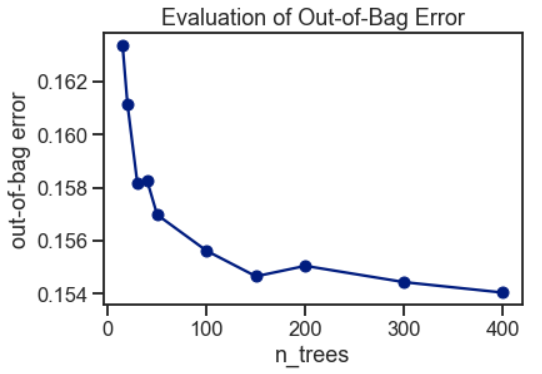The out-of-bag error appeared to have stabilized around 150 trees. 袋外误差似乎已稳定在150棵树附近。
# Create the model with 150 trees
forest = RandomForestClassifier(n_estimators=150, random_state=1, n_jobs=-1)

# Fit training data and training labels to forest
forest.fit(X_train_bopt, y_train_bopt)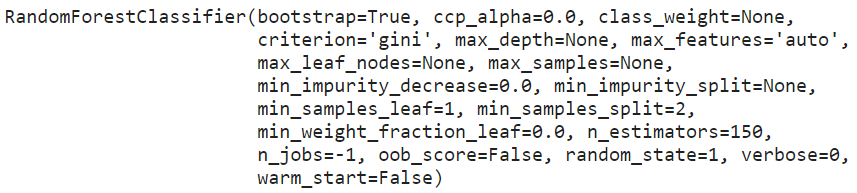n_nodes = []
max_depths = []

for ind_tree in forest.estimators_:
n_nodes.append(ind_tree.tree_.node_count)
max_depths.append(ind_tree.tree_.max_depth)

print(f'Random Forest has an average number of nodes {int(np.mean(n_nodes))} with an average maximum depth of {int(np.mean(max_depths))}.')

print(f'Model Accuracy for train data: {forest.score(X_train_bopt, y_train_bopt)}')
print(f'Model Accuracy for test data: {forest.score(X_test_bopt, y_test_bopt)}')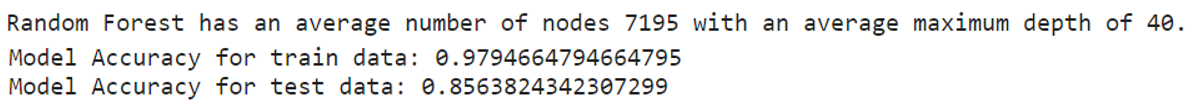From the above, each decision tree in the random forest has many nodes and is extremely deep. Although each individual decision tree may overfit to a particular subset of the training data, the use of random forest had produced a slightly higher accuracy score for the test data. 综上所述，随机森林中的每个决策树都有许多节点，并且深度非常大。 尽管每个决策树都可能过度适合训练数据的特定子集，但是使用随机森林对测试数据的准确性得分略高。
功能重要性 (Feature Importance)
The feature importance of each feature of the dataset can be obtained by using the feature importance property of the model. Feature importance gives a score for each feature of the data. The higher the score, the more important or relevant the feature is towards the target variable. 可以通过使用模型的特征重要性属性来获得数据集的每个特征的特征重要性。 特征重要性为数据的每个特征给出分数。 分数越高，特征对目标变量的重要性或相关性就越高。
Feature importance is an in-built class that comes with Tree-Based Classifiers. We have used the decision tree and random forest to rank the feature importance for the dataset. 功能重要性是基于树的分类器附带的内置类。 我们已经使用决策树和随机森林对数据集的特征重要性进行排序。
feature_imp = pd.Series(tree.feature_importances_,
index=X.columns).sort_values(ascending=False)

ax = feature_imp.plot(kind='bar')
ax.set(title='Feature Importance - Decision Trees',
ylabel='Relative Importance');

feature_imp = pd.Series(forest.feature_importances_,
index=X.columns).sort_values(ascending=False)

ax = feature_imp.plot(kind='bar')
ax.set(title='Feature Importance - Random Forest',
ylabel='Relative Importance');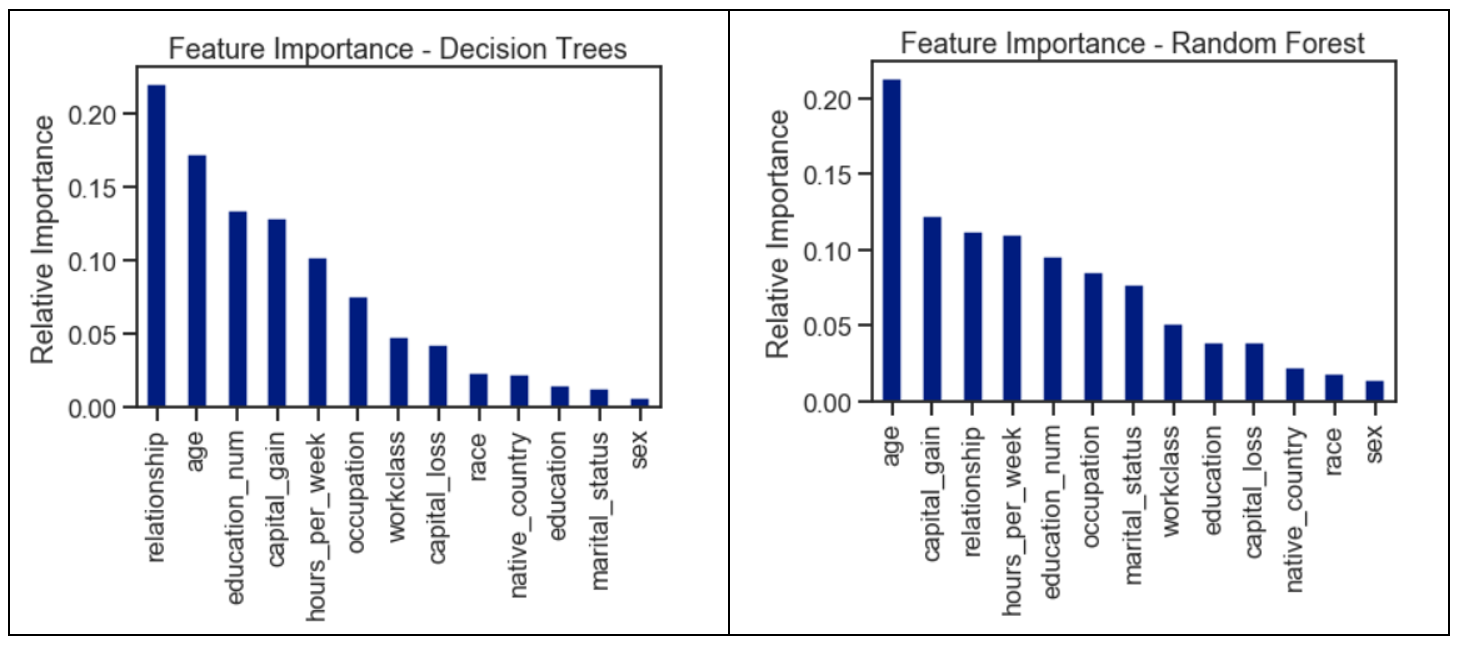The features were ranked based on their importance considered by the respective classifiers. The values were computed by summing the reduction in Gini Impurity over all of the nodes of the tree in which the feature is used. 根据各个分类器考虑的重要性对功能进行排名。 通过对使用该特征的树的所有节点上的基尼杂质减少量求和来计算这些值。
使用2种方法进行特征选择： (Feature Selection using 2 Methods:)
1.单变量选择 (1. Univariate Selection)
Statistical tests can be used to select those features that have the strongest relationship with the target variable. The scikit-learn library provides the SelectKBest class to be used with a suite of different statistical tests to select a specific number of features. We used the chi-squared (chi²) statistical test for non-negative features to select 10 of the best features from the dataset. 可以使用统计检验来选择与目标变量关系最密切的那些特征 。 scikit-learn库提供SelectKBest类，该类将与一组不同的统计测试一起使用，以选择特定数量的功能。 我们使用非负特征的卡方(chi²)统计检验从数据集中选择10个最佳特征。
# Apply SelectKBest class to extract top 10 best features
bestfeatures = SelectKBest(score_func=chi2, k=10)
fit = bestfeatures.fit(X,y)
dfscores = pd.DataFrame(fit.scores_)
dfcolumns = pd.DataFrame(X.columns)

# Concatenate two dataframes for better visualization
featureScores = pd.concat([dfcolumns,dfscores],axis=1)
featureScores.columns = ['Features','Score']  # naming the dataframe columns
print(featureScores.nlargest(10,'Score'))  # print 10 best features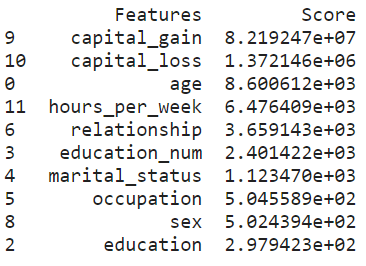2.具有热图的相关矩阵 (2. Correlation Matrix with Heat Map)
Correlation states how the features are related to each other or the target variable. Correlation can be positive (increase in one value of feature increases the value of the target variable) or negative (increase in one value of feature decreases the value of the target variable). A heat map makes it easy to identify which features are most related to the target variable. 关联说明要素之间如何相互关联或与目标变量关联。 相关可以是正的(增加一个特征值增加目标变量的值)或负的(增加一个特征值减少目标变量的值)。 通过热图 ，可以轻松识别出哪些特征与目标变量最相关 。
# Obtain correlations of each features in dataset
sns.set(font_scale=1.4)
corrmat = clean_df.corr()
top_corr_features = corrmat.index
plt.figure(figsize=(30,30))

# Plot heat map
correlation = sns.heatmap(clean_df[top_corr_features].corr(),annot=True,fmt=".3f",cmap='Blues')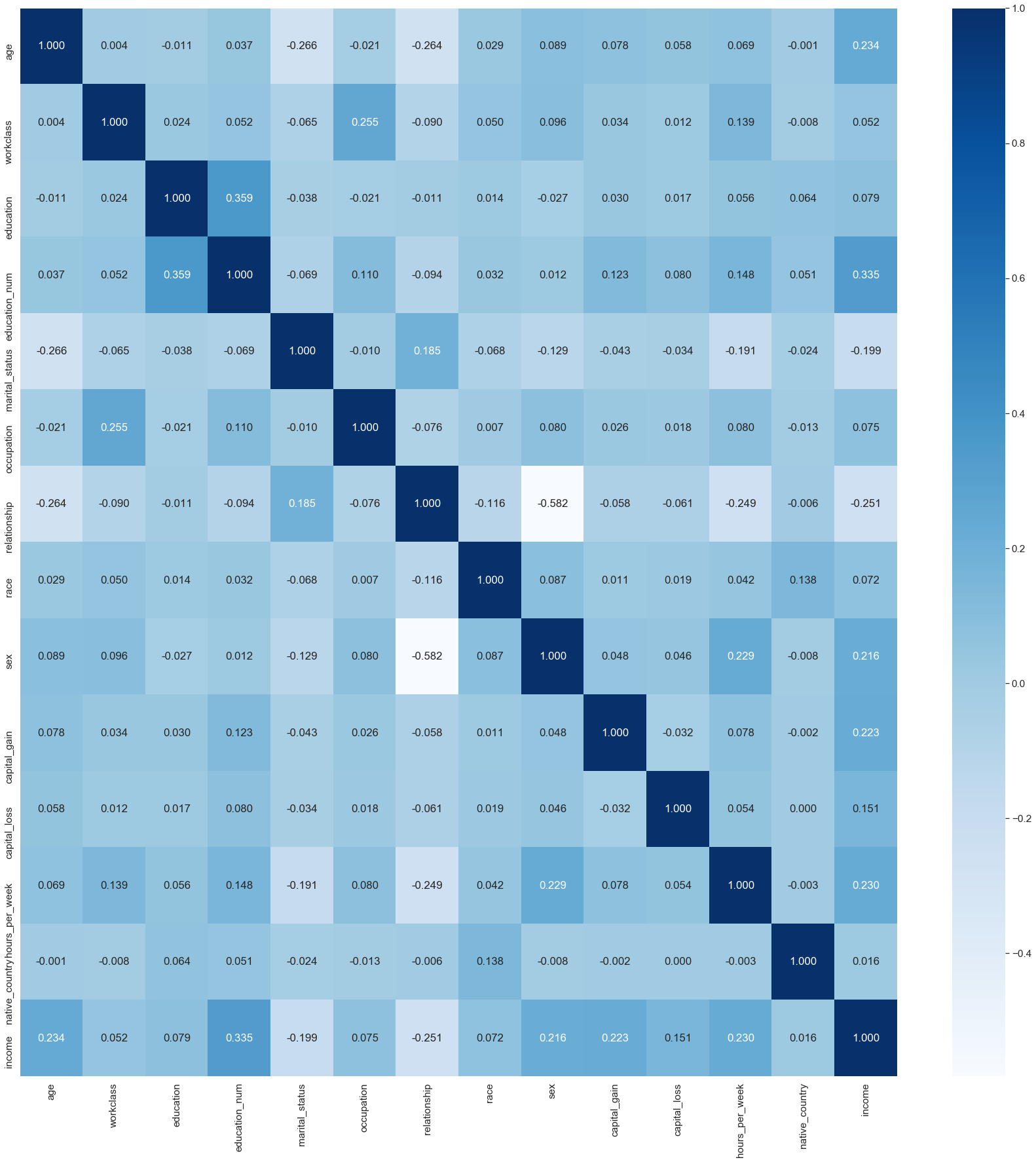上采样 (Upsampling)
Upsampling is the process of randomly duplicating observations from the minority class in order to reinforce its signal. There are several heuristics for doing so, but the most common way is to simply resample with replacement. 上采样是随机复制少数群体的观察结果以增强其信号的过程 。 这样做有几种启发式方法，但是最常见的方法是简单地用替换进行重新采样。
# Separate majority and minority classes
df_majority = clean_df[clean_df.income==0]
df_minority = clean_df[clean_df.income==1]

# Upsample minority class
df_minority_upsampled = resample(df_minority,
replace=True,     # sample with replacement
n_samples=24720,  # to match majority class
random_state=1)   # reproducible results

# Combine majority class with upsampled minority class
df_upsampled = pd.concat([df_majority, df_minority_upsampled])

# Display new class counts
df_upsampled.income.value_counts()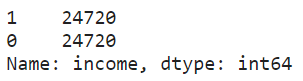df_upsampled.income.value_counts(normalize=True)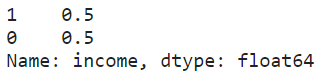Now that the dataset has been balanced, we are ready to split and scale this dataset for training and testing using the optimized random forest model. 现在数据集已经达到平衡，我们已经准备好使用优化的随机森林模型拆分和缩放该数据集，以进行训练和测试。
X_upsamp = df_upsampled[feature_cols]
y_upsamp = df_upsampled['income']

X_train, X_test, y_train, y_test = train_test_split(X_upsamp, y_upsamp,
test_size = 0.3,
random_state = 1)

# Perform pre-processing to scale numeric features
scale = preprocessing.StandardScaler()
X_train = scale.fit_transform(X_train)

# Test features are scaled using the scaler computed for the training features
X_test = scale.transform(X_test) 通过网格搜索进行随机森林优化 (Random Forest Optimization through Grid Search)
Grid search is an exhaustive search over specified parameter values for an estimator. It selects combinations of hyperparameters from a grid, evaluates them using cross-validation on the training data, and returns the values that perform the best. 网格搜索是对估计器的指定参数值的详尽搜索 。 它从网格中选择超参数组合，对训练数据使用交叉验证对它们进行评估，然后返回性能最佳的值。
We have selected the following model parameters for the grid search: 我们为网格搜索选择了以下模型参数 ：
n_estimators: The number of trees in the forest. n_estimators：森林中树木的数量。 max_depth: The maximum depth of the tree. max_depth：树的最大深度。 min_samples_split: The minimum number of samples required to split an internal node. min_samples_split：拆分内部节点所需的最小样本数。 # Set the model parameters for grid search
model_params = {'n_estimators': [150, 200, 250, 300],
'max_depth': [15, 20, 25],
'min_samples_split': [2, 4, 6]}

# Create random forest classifier model
rf_model = RandomForestClassifier(random_state=1)

# Set up grid search meta-estimator
gs = GridSearchCV(rf_model, model_params,n_jobs=-1, scoring='roc_auc', cv=3)

# Train the grid search meta-estimator to find the best model
best_model = gs.fit(X_train, y_train)

# Print best set of hyperparameters
from pprint import pprint
pprint(best_model.best_estimator_.get_params())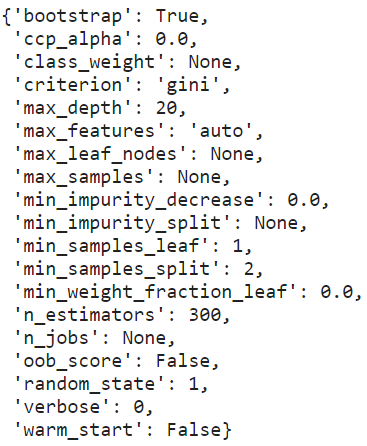Based on the grid search, the best hyperparameter values were not the defaults. This shows the importance of tuning a model for a specific dataset. Each dataset will have different characteristics, and the model that does best on one dataset will not necessarily do the best across all datasets. 基于网格搜索，最佳超参数值不是默认值。 这表明为特定数据集调整模型的重要性。 每个数据集将具有不同的特征，并且在一个数据集上表现最佳的模型不一定会在所有数据集上表现最佳。
使用最佳模型优化随机森林 (Use the Best Model to Optimize Random Forest)
n_nodes = []
max_depths = []

for ind_tree in best_model.best_estimator_:
n_nodes.append(ind_tree.tree_.node_count)
max_depths.append(ind_tree.tree_.max_depth)

print(f'The optimized random forest has an average number of nodes {int(np.mean(n_nodes))} with an average maximum depth of {int(np.mean(max_depths))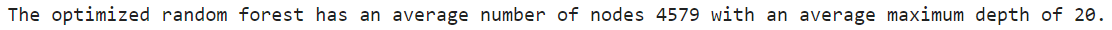The best maximum depth was not unlimited, this indicates that restricting the maximum depth of the individual decision trees can improve the cross validation performance of the random forest. 最佳最大深度不是无限的，这表明限制单个决策树的最大深度可以提高随机森林的交叉验证性能。
print(f'Model Accuracy for train data: {best_model.score(X_train, y_train)}')
print(f'Model Accuracy for test data: {best_model.score(X_test, y_test)}')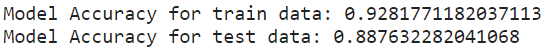Although the performance achieved by the optimized model was slightly below that of the decision tree and default model, the gap between the model accuracy obtained for both the train data and test data was minimized (~4%). This represents a good fit of the learning curve where a high accuracy rate was achieved by using the trained model on the test data. 尽管通过优化模型获得的性能略低于决策树和默认模型，但是针对火车数据和测试数据获得的模型精度之间的差距已最小化(〜4％)。 这代表了学习曲线的良好拟合，其中通过在测试数据上使用经过训练的模型可以实现较高的准确率。
模型的性能评估 (Performance Evaluation of Models)
# Predict target variables (ie. labels) for each classifer
dt_classifier_name = ["Decision Tree"]
dt_predicted_labels = tree.predict(X_test_bopt)

rf_classifier_name = ["Random Forest"]
rf_predicted_labels = forest.predict(X_test_bopt)

best_model_classifier_name = ["Optimized Random Forest"]
best_model_predicted_labels = best_model.predict(X_test) 1.分类报告 (1. Classification Report)
The classification report shows a representation of the main classification metrics on a per-class basis and gives a deeper intuition of the classifier behavior over global accuracy, which can mask functional weaknesses in one class of a multi-class problem. The metrics are defined in terms of true and false positives, and true and false negatives. 分类报告显示了每个分类的主要分类指标，并给出了分类器行为相对于全局准确性的更直观认识，这可以掩盖一类多分类问题中的功能弱点。 度量是根据正确和错误肯定以及正确和错误否定来定义的。
Precision is the ability of a classifier not to label an instance positive that is actually negative. For each class, it is defined as the ratio of true positives to the sum of true and false positives. 精度是分类器不标记实际为负的实例正的能力。 对于每个类别，它定义为真阳性与真假阳性之和的比率。
For all instances classified positive, what percent was correct? 对于所有归类为阳性的实例，正确的百分比是多少？ Recall is the ability of a classifier to find all positive instances. For each class, it is defined as the ratio of true positives to the sum of true positives and false negatives. 回忆是分类器查找所有正实例的能力。 对于每个类别，它定义为真阳性与真阳性与假阴性总和之比。
For all instances that were actually positive, what percent was classified correctly? 对于所有实际为正的实例，正确分类的百分比是多少？ The F1-score is a weighted harmonic mean of precision and recall such that the best score is 1.0 and the worst is 0.0. Generally, F1-scores are lower than accuracy measures as they embed precision and recall into their computation. As a rule of thumb, the weighted average of the F1-score should be used to compare classifier models, not global accuracy. F1分数是精确度和召回率的加权谐波平均值，因此最佳分数是1.0，最差分数是0.0。 通常，F1分数将精度和召回率嵌入到计算中，因此它们比精度度量要低。 根据经验，应该使用F1分数的加权平均值来比较分类器模型，而不是整体精度。
Support is the number of actual occurrences of the class in the specified dataset. Imbalanced support in the training data may indicate structural weaknesses in the reported scores of the classifier and could indicate the need for stratified sampling or re-balancing. Support does not change between models but instead diagnoses the evaluation process. 支持是指定数据集中该类的实际出现次数。 训练数据中支持不平衡可能表明分类器报告的分数存在结构性缺陷，并且可能表明需要分层抽样或重新平衡。 支持在模型之间不会改变，而是诊断评估过程。
print("Classification Report for",dt_classifier_name, " :\n ",
metrics.classification_report(y_test_bopt, dt_predicted_labels,
target_names=['Income <= U$50K','Income > U$50K']))

print("Classification Report for ",rf_classifier_name, " :\n ",
metrics.classification_report(y_test_bopt, rf_predicted_labels,
target_names=['Income <= U$50K','Income > U$50K']))

print("Classification Report for ",best_model_classifier_name, " :\n ",
metrics.classification_report(y_test,best_model_predicted_labels,
target_names=['Income <= U$50K','Income > U$50K']))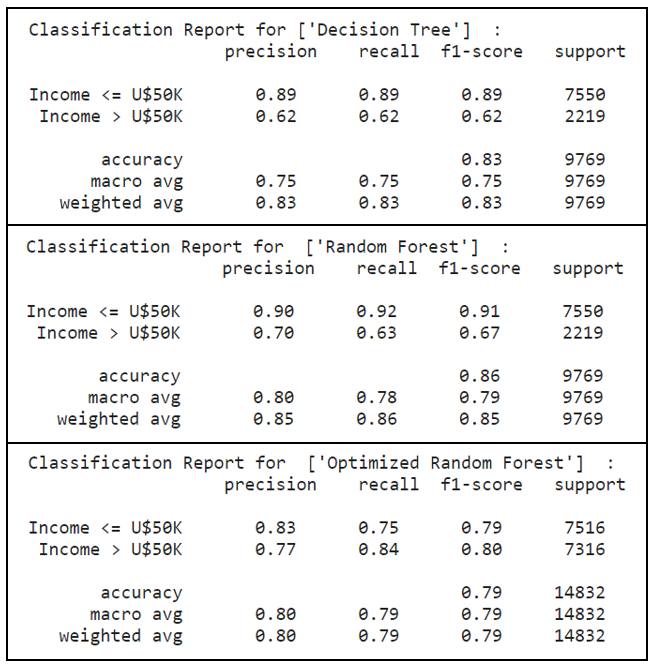The optimized random forest has performed well in the above metrics. In particular, with upsampling performed to maintain a balanced dataset, a significant observation was noted in the minority class (ie. label ‘1’ representing income > U$50K), where recall scores had improved 35%, from 0.62 to 0.84, by using the optimized random forest model. 经过优化的随机森林在上述指标中表现良好。 尤其是，为了保持数据集的平衡而进行了上采样， 在少数族裔类别中观察到了显着的结果(即标签“ 1”代表收入> 5万美元)， 召回得分提高了35％，从0.62提高到0.84，使用优化的随机森林模型。 With a higher precision and recall scores, the optimized random forest model was able to correctly label instances that were indeed positive. Out of these instances which were actually positive, the optimized random forest model had classified them correctly to a large extent. This directly translates into a higher F1-score as a weighted harmonic mean of precision and recall. 优化的随机森林模型具有更高的精度和召回得分 ，能够正确标记确实为阳性的实例。 在这些实际为阳性的实例中，优化后的随机森林模型在很大程度上将它们正确分类。 这直接转化为更高的F1得分，作为精确度和召回率的加权谐波平均值。 2.混淆矩阵 (2. Confusion Matrix) The confusion matrix takes a fitted scikit-learn classifier and a set of test x and y values and returns a report showing how each of the test values predicted classes compare to their actual classes. These provide similar information as what is available in a classification report, but rather than top-level scores, they provide deeper insight into the classification of individual data points. 混淆矩阵采用适合的scikit-learn分类器和一组测试x和y值，并返回报告，显示每个预测值预测类与实际类的比较。 这些提供的信息与分类报告中提供的信息类似，但是它们不是顶级分数，而是提供了对单个数据点分类的更深入了解。 print("Confusion Matrix for",dt_classifier_name) skplt.metrics.plot_confusion_matrix(y_test_bopt, dt_predicted_labels, normalize=True) plt.show() print("Confusion Matrix for",rf_classifier_name) skplt.metrics.plot_confusion_matrix(y_test_bopt, rf_predicted_labels, normalize=True) plt.show() print("Confusion Matrix for",best_model_classifier_name) skplt.metrics.plot_confusion_matrix(y_test, best_model_predicted_labels, normalize=True) plt.show()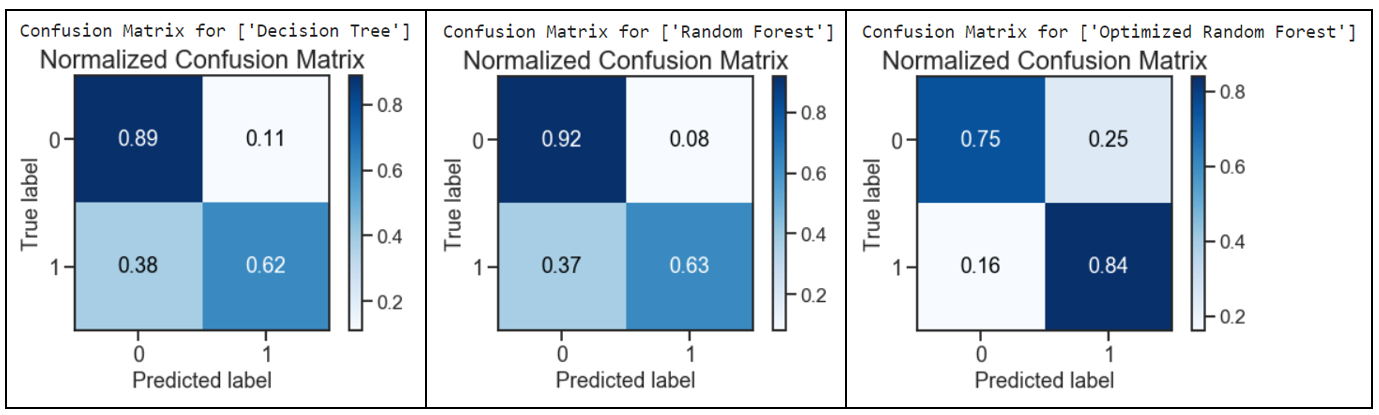The optimized random forest had performed well with a decrease in the Type 2 Error: False Negatives (predicted income <= U$50K but actually income > U$50K). A remarkable decrease of 58% was obtained from a score of 0.38 to 0.16 when comparing the results for decision tree against the optimized random forest. 经过优化的随机森林表现良好，并且减少了Type 2错误：False Negatives (预期收入<= 5万美元，但实际收入> 5万美元)。 将决策树的结果与优化的随机森林进行比较时， 得分从0.38下降到0.16 ， 下降了58％ 。 However, the Type 1 Error: False Positives (predicted > U$50K but actually <= U\$50K) had approximately tripled, from 0.08 to 0.25, by comparing the optimized random forest with the default random forest model. 但是，通过将优化后的随机森林与默认随机森林模型进行比较， 类型1错误：误报 (预测为> 5万美元，但实际<= 5万美元) 大约增加了三倍，从0.08到0.25 。
Overall, the impact of having more false positives was mitigated with a notable decrease in false negatives. With a good outcome of the test values predicted classes as compared to their actual classes, the confusion matrix results for the optimized random forest had outperformed the other models. 总体而言，误报率明显下降，减轻了更多误报率的影响。 测试值预测类比其实际类具有更好的结果，优化随机森林的混淆矩阵结果优于其他模型。
3.精确调用曲线 (3. Precision-Recall Curve)
Precision-Recall curve is a metric used to evaluate a classifier’s quality. The precision-recall curve shows the trade-off between precision, a measure of result relevancy, and recall, a measure of how many relevant results are returned. A large area under the curve represents both high recall and precision, the best-case scenario for a classifier, showing a model that returns accurate results for the majority of classes it selects. 精确召回曲线是用于评估分类器质量的指标。 精度调用曲线显示了精度(即结果相关性的度量)和召回率(即返回了多少相关结果的度量)之间的权衡。 曲线下的较大区域代表了较高的查全率和精度，这是分类器的最佳情况，它显示了一个模型，该模型针对选择的大多数类别返回准确的结果。
fig, axList = plt.subplots(ncols=3)
fig.set_size_inches(21,6)

# Plot the Precision-Recall curve for Decision Tree
ax = axList
dt_predicted_proba = tree.predict_proba(X_test_bopt)
precision, recall, _ = precision_recall_curve(y_test_bopt, dt_predicted_proba[:,1])
ax.plot(recall, precision,color='black')
ax.set(xlabel='Recall', ylabel='Precision', xlim=[0, 1], ylim=[0, 1],
title='Precision-Recall Curve - Decision Tree')
ax.grid(True)

# Plot the Precision-Recall curve for Random Forest
ax = axList
rf_predicted_proba = forest.predict_proba(X_test_bopt)
precision, recall, _ = precision_recall_curve(y_test_bopt, rf_predicted_proba[:,1])
ax.plot(recall, precision,color='green')
ax.set(xlabel='Recall', ylabel='Precision', xlim=[0, 1], ylim=[0, 1],
title='Precision-Recall Curve - Random Forest')
ax.grid(True)

# Plot the Precision-Recall curve for Optimized Random Forest
ax = axList
best_model_predicted_proba = best_model.predict_proba(X_test)
precision, recall, _ = precision_recall_curve(y_test, best_model_predicted_proba[:,1])
ax.plot(recall, precision,color='blue')
ax.set(xlabel='Recall', ylabel='Precision', xlim=[0, 1], ylim=[0, 1],
title='Precision-Recall Curve - Optimised Random Forest')
ax.grid(True)
plt.tight_layout()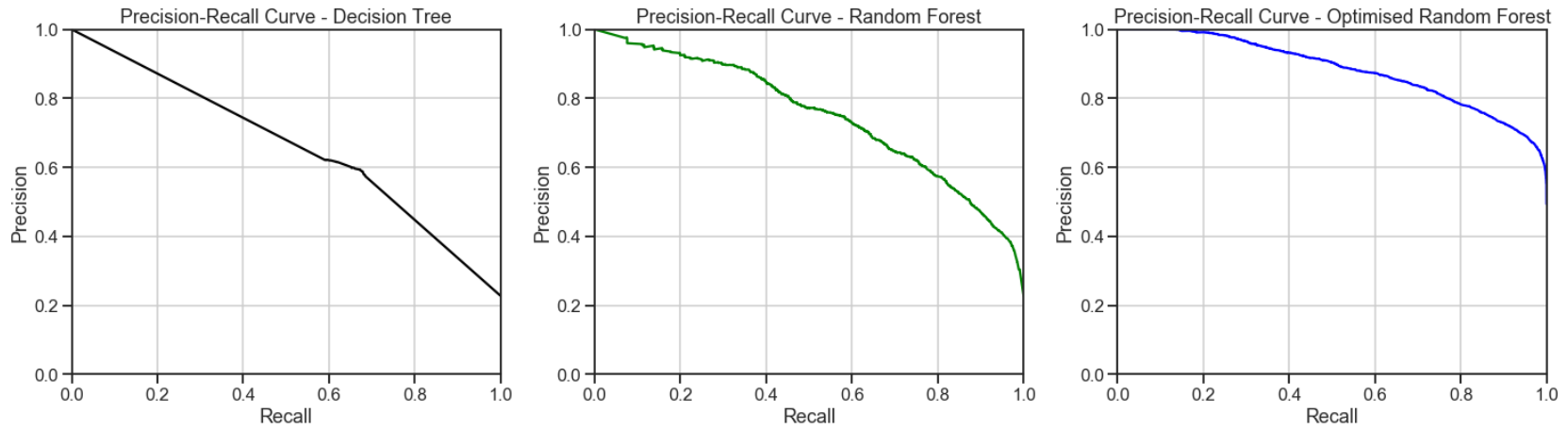The optimized random forest classifier achieved a higher area under the precision-recall curve. This represents high recall and precision scores, where high precision relates to a low false-positive rate, and a high recall relates to a low false-negative rate. High scores in both showed that the optimized random forest classifier had returned accurate results (high precision), as well as a majority of all positive results (high recall). 优化的随机森林分类器在精确召回曲线下获得了更大的面积。 这代表了较高的查全率和精确度分数，其中高精度与低假阳性率相关，而高查全率与较低的假阴性率相关。 两者均获得高分，表明优化后的随机森林分类器已返回准确结果(高精度)，以及大部分积极结果(高召回率)。
4. ROC曲线和AUC (4. ROC Curve and AUC)
A Receiver Operating Characteristic (“ROC”)/Area Under the Curve (“AUC”) plot allows the user to visualize the trade-off between the classifier’s sensitivity and specificity. 接收器工作特征(“ ROC”)/曲线下面积(“ AUC”)曲线图使用户可以直观地看到分类器的灵敏度和特异性之间的权衡。
The ROC is a measure of a classifier’s predictive quality that compares and visualizes the trade-off between the model’s sensitivity and specificity. When plotted, a ROC curve displays the true positive rate on the Y axis and the false positive rate on the X axis on both a global average and per-class basis. The ideal point is therefore the top-left corner of the plot: false positives are zero and true positives are one. ROC是对分类器预测质量的一种度量，它比较并可视化模型的敏感性和特异性之间的权衡。 绘制时，ROC曲线在全局平均值和每个类别的基础上，在Y轴上显示真实的阳性率，在X轴上显示假的阳性率。 因此理想点是图的左上角：假阳性为零，真阳性为一。
AUC is a computation of the relationship between false positives and true positives. The higher the AUC, the better the model generally is. However, it is also important to inspect the “steepness” of the curve, as this describes the maximization of the true positive rate while minimizing the false positive rate. AUC是假阳性和真阳性之间的关系的计算。 AUC越高，模型通常越好。 但是，检查曲线的“陡度”也很重要，因为这描述了真实阳性率的最大化，同时最小化了阳性阳性率。
fig, axList = plt.subplots(ncols=3)
fig.set_size_inches(21,6)

# Plot the ROC-AUC curve for Decision Tree
ax = axList
dt = tree.fit(X_train_bopt, y_train_bopt.values.ravel())
dt_predicted_label_r = dt.predict_proba(X_test_bopt)

def plot_auc(y, probs):
fpr, tpr, threshold = roc_curve(y, probs[:,1])
auc = roc_auc_score(y_test_bopt, dt_predicted_labels)
ax.plot(fpr, tpr, color = 'black', label = 'AUC_Decision Tree = %0.2f' % auc)
ax.plot([0, 1], [0, 1],'r--')
ax.legend(loc = 'lower right')
ax.set(xlabel='False Positive Rate',
ylabel='True Positive Rate',
xlim=[0, 1], ylim=[0, 1],
title='ROC curve')

plot_auc(y_test_bopt, dt_predicted_label_r)
ax.grid(True)

# Plot the ROC-AUC curve for Random Forest
ax = axList
rf = forest.fit(X_train_bopt, y_train_bopt.values.ravel())
rf_predicted_label_r = rf.predict_proba(X_test_bopt)

def plot_auc(y, probs):
fpr, tpr, threshold = roc_curve(y, probs[:,1])
auc = roc_auc_score(y_test_bopt, rf_predicted_labels)
ax.plot(fpr, tpr, color = 'green', label = 'AUC_Random Forest = %0.2f' % auc)
ax.plot([0, 1], [0, 1],'r--')
ax.legend(loc = 'lower right')
ax.set(xlabel='False Positive Rate',
ylabel='True Positive Rate',
xlim=[0, 1], ylim=[0, 1],
title='ROC curve')

plot_auc(y_test_bopt, rf_predicted_label_r);
ax.grid(True)

# Plot the ROC-AUC curve for Optimized Random Forest
ax = axList
best_model = best_model.fit(X_train, y_train.values.ravel())
best_model_predicted_label_r = best_model.predict_proba(X_test)

def plot_auc(y, probs):
fpr, tpr, threshold = roc_curve(y, probs[:,1])
auc = roc_auc_score(y_test, best_model_predicted_labels)
ax.plot(fpr, tpr, color = 'blue', label = 'AUC_Optimised Random Forest = %0.2f' % auc)
ax.plot([0, 1], [0, 1],'r--')
ax.legend(loc = 'lower right')
ax.set(xlabel='False Positive Rate',
ylabel='True Positive Rate',
xlim=[0, 1], ylim=[0, 1],
title='ROC curve')

plot_auc(y_test, best_model_predicted_label_r);
ax.grid(True)
plt.tight_layout()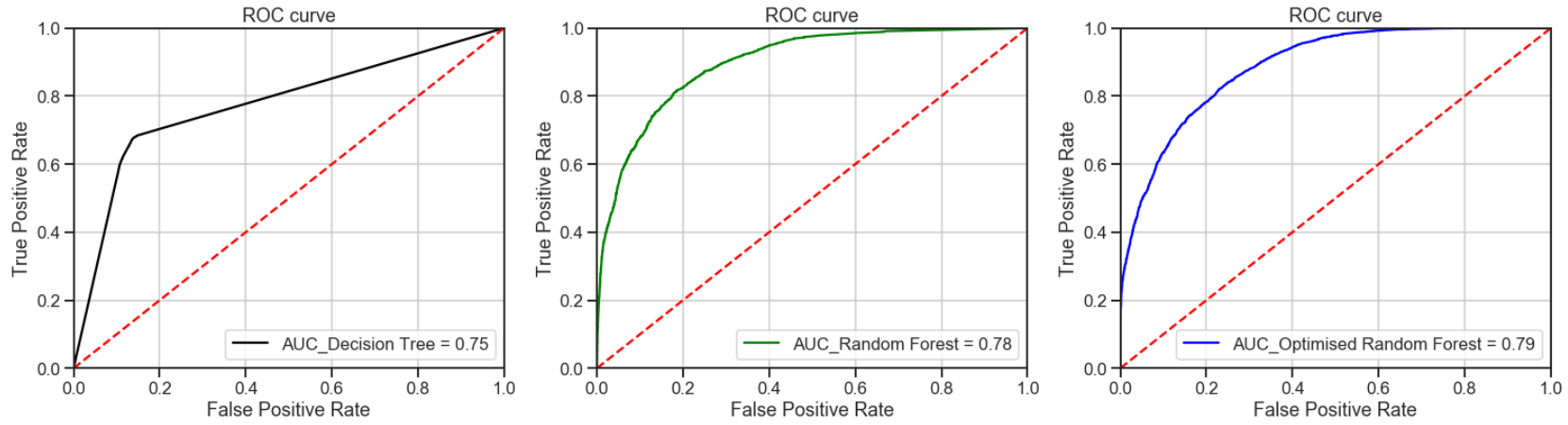All the models had outperformed the baseline guess with the optimized random forest achieving the best AUC results. Thus, indicating that the optimized random forest is a better classifier. 所有模型均优于基线猜测，优化的随机森林获得了最佳的AUC结果。 因此，表明优化的随机森林是更好的分类器。
5.校准曲线 (5. Calibration Curve)
When performing classification, one often wants to predict not only the class label, but also the associated probability. This probability gives some kind of confidence on the prediction. Thus, the calibration plot is useful for determining whether predicted probabilities can be interpreted directly as an confidence level. 在进行分类时，人们经常不仅要预测分类标签，还要预测相关的概率。 这种可能性使预测具有某种信心。 因此，校准图可用于确定预测的概率是否可以直接解释为置信度。
# Plot calibration curves for a set of classifier probability estimates.
tree = DecisionTreeClassifier()
forest = RandomForestClassifier()

tree_probas = tree.fit(X_train_bopt, y_train_bopt).predict_proba(X_test_bopt)
forest_probas = forest.fit(X_train_bopt, y_train_bopt).predict_proba(X_test_bopt)

probas_list = [tree_probas, forest_probas]
clf_names = ['Decision Tree','Random Forest']

skplt.metrics.plot_calibration_curve(y_test_bopt, probas_list, clf_names,figsize=(10,6))
plt.show()# Plot calibration curves for a set of classifier probability estimates.
best_model = RandomForestClassifier()

best_model_probas = best_model.fit(X_train, y_train).predict_proba(X_test)

probas_list = [best_model_probas]
clf_names = ['Optimized Random Forest']

skplt.metrics.plot_calibration_curve(y_test, probas_list, clf_names, cmap='winter', figsize=(10,6))
plt.show()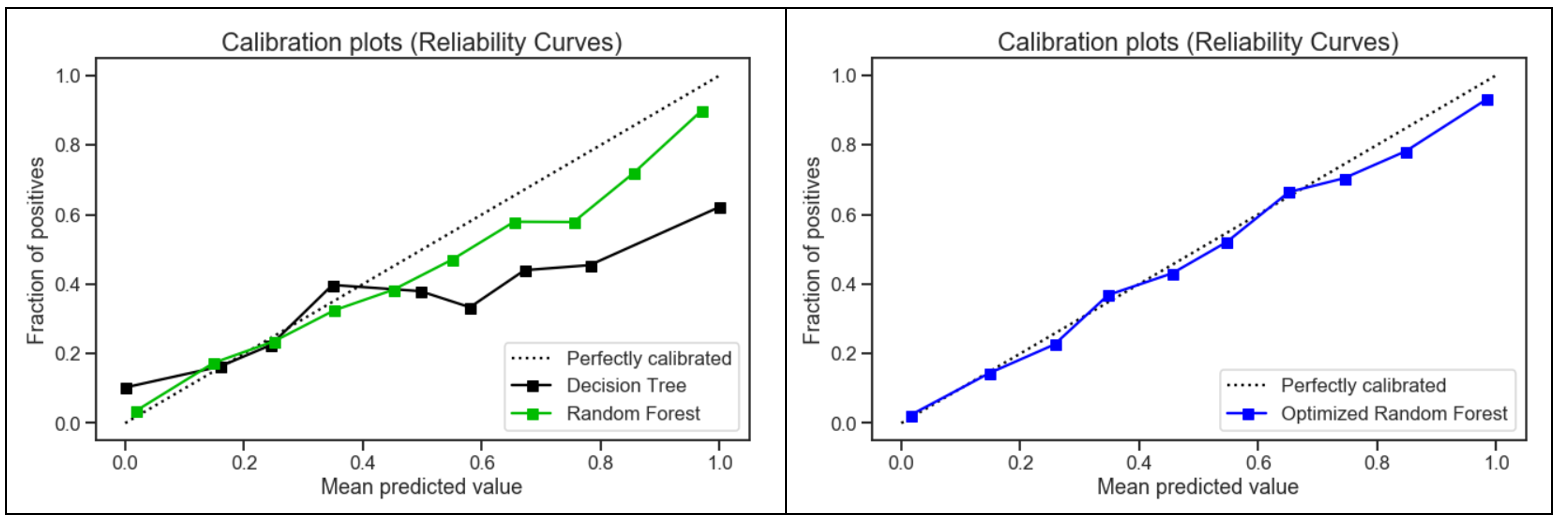Compared to the other two models, the calibration plot for the optimized random forest was the closest to being perfectly calibrated. Hence, the optimized random forest was more reliable and better able to generalize to new data. 与其他两个模型相比，优化后的随机森林的校准图最接近于完美校准。 因此，优化后的随机森林更加可靠，能够更好地推广到新数据。
结论 (Conclusion)
The optimized random forest had a better generalization performance on the testing set with reduced variance as compared to the other models. Decision trees tend to overfit and pruning helped to reduce variance to a point. The random forest addressed the shortcomings of decision trees with a strong modeling technique which was more robust than a single decision tree. 与其他模型相比， 优化后的随机森林在测试集上具有更好的泛化性能 ，并且方差减小。 决策树倾向于过度拟合，而修剪有助于将方差降低到一定程度。 随机森林使用强大的建模技术解决了决策树的缺点，该技术比单个决策树更强大。
The use of optimization for random forest had a significant impact on the results with the following 3 factors being considered: 对随机森林的优化使用对结果有重大影响，考虑了以下三个因素：
Feature selection to chose the ideal number of features to prevent overfitting and improve model interpretability 选择特征以选择理想数量的特征，以防止过度拟合并提高模型的可解释性 Upsampling of the minority class to create a balanced dataset 少数类的上采样以创建平衡的数据集 Grid search to select the best hyper-parameters to maximize model performance 网格搜索以选择最佳超参数以最大化模型性能 Lastly, the results were also attributed by the unique quality of random forest, where it adds additional randomness to the model while growing the trees. Instead of searching for the most important feature while splitting a node, it searches for the best feature among a random subset of features. This results in a wide diversity that generally results in a better model for classification problems. 最后，结果还归因于随机森林的独特质量，即在树木生长时为模型增加了额外的随机性。 它不是在分割节点时搜索最重要的特征，而是在特征的随机子集中搜索最佳特征 。 这导致了广泛的多样性，通常可以为分类问题提供更好的模型。
翻译自: https://medium.com/towards-artificial-intelligence/use-of-decision-trees-and-random-forest-in-machine-learning-1e35e737b638机器学习中决策树的随机森林
展开全文python 人工智能
• 下面介绍下机器学习理论中最为重要的“决策树”部分。个人感觉决策树弄懂了，你就懂得了机器学习的30%理论！1：梯度迭代决策树（gradient booster decision tree） 这种决策树只是一种回归树，不存在分类树。在回归...
• ## 机器学习之决策树

千次阅读 2017-03-26 13:36:58
在之前说了用线性回归的方法来对训练数据进行训练，然后通过得到的方程式来对测试数据进行了测试...对数据使用决策树的方法对鸢尾花进行分类，进行实验比较、精度比较并写成实验报告。 二、 实验原理 决策树(Decision
• 文章目录1 决策树算法简介2 决策树算法2.1 引例例1例22.2 算法分类(1) 信息熵(2) 信息增益(3) 增益率(4) 基尼指数3 决策树算法优缺点4 实验参考资料 1 决策树算法简介 决策树(Decision Tree) 是在已知各种情况发生...
• 这次，我们要做一个真正的机器学习算法，决策树算法。当然，它也是一个多元分类器。相比较K近邻算法对于数值型的数据处理较为舒服，因为毕竟是算距离，所以你就算是跑到天涯海角，也能算出来。但是决策树对于数值型...数据 数学
• [机器学习]决策树算法的MATLAB实现 这是一篇关于决策树算法的MATLAB实现的文章，也是我的课堂实验，学习的书籍为西瓜书。此文章包含树的建立(使用信息增益，基尼指数)，绘图，预测以及剪枝（后剪枝），部分代码为...matlab
• 关闭 我的博客 防冷涂的辣 ... 2017之区块链技术实战线上峰会 程序员9月书讯 每周荐书：ES6、虚拟现实、物联网（评论送书） ...机器学习(周志华) 参考答案 第四章 决策树
• 决策树是一种机器学习的方法。决策树的生成算法有ID3, C4.5和C5.0等。决策树是一种树形结构，其中每个内部节点表示一个属性上的判断，每个分支代表一个判断结果的输出，最后每个叶节点代表一种分类结果。 机器学习...python 数据挖掘
• 为了验证每个算法在每种不同样本数量的能力，就做了一下实验，本文将的是决策树在文本算法中的精准率。 效果图 先看一下没有任何调参的情况下的效果吧！ 通过以上数据可以看出决策树在样本数量较低的情况下还...python
• 决策树简介决策树是常见的机器学习算法之一。主要用于分类和回归。是一种非参数的监督式学习方法。 决策树中的几个词： 属性、特征、属性选择度量、属性特征的拓扑结构、分裂属性特征。python 算法
• 决策树可以使用不熟悉的数据集合，并从中提取出一系列规则，机器学习算法最终将使用这些从数据集中创造的规则。决策树的优点为：计算复杂度不高，输出结果易于理解，对中间值的缺失不敏感，可以处理不相关特征数据。...python ID3 过拟合
• 该节主要是把《机器学习实战》书上第三章关于决策树的相关代码照样子实现了一遍。对其中一些内容作了些补充，对比ID3与C45区别，同时下载了一个大样本集实验决策树的准确率。首先，对于决策树的原理，很多很好的博客...python
• 什么是决策树呢？其实很直观，这样的就是  不说了，先看数据：  这是一个医疗检测的数据，前面六个是指标，具体是什么其实没有意义，说的好像化验单上的那些医学术语你都知道似得。最后一个就是结果。我们...Python
• 决策树学习的重点是根据训练...学习决策树的一个基本点在于计算信息增益。具体计算方法详见《机器学习》P40 3.4.1《哪个属性是最佳的分类属性》中的例子。  写的很好的一个博客算法杂货铺——分类算法之决策树
• 一、什么是决策树 本章着重对算法部分进行讲解，原理部分不过多叙述，有兴趣的小伙伴可以自行查阅其他文献/文章 决策树(decision tree)是一种基本的分类与回归方法。决策树模型呈树形结构，在分类问题中，表示基于...python 人工智能
• 最近在搞一些关于机器学习的小东西，其中有一部分就是关于决策树的。过程中遇到了一些小问题，现记录并与大家分享。 一、问题描述：使用西瓜数据集构建决策树，并将构建的决策树进行可视化操作。 二、问题简析：首先...python
• 上一篇机器学习的博客我详细说了机器学习决策树算法的原理，这篇博客我就以一个小例子来说明机器学习决策树算法的实现。用Python实现机器学习中的决策树算法需要用到机器学习的库，sklearn，我的博客有详细讲解...算法
• 机器学习中，决策树是一个预测模型；他代表的是对象属性与对象值之间的一种映射关系。树中每个节点表示某个对象，而每个分叉路径则代表的某个可能的属性值，而每个叶结点则对应从根节点到该叶节点所经历的路径所表示...python id3算法 数据挖掘
• 　决策树学习是以实例为基础的归纳学习 　决策树学习采用的是自顶向下的递归方法，其基本思想是以信息熵为度量构造一棵熵值下降最快的树。到叶子节点的处的熵值为零，此时每个叶结点中的实例都属于同一类。 1....
• 决策树学习比较典型的有三种算法：ID3 C4.5 CART。 决策树是一种分类预测算法，通过训练样本建立的决策树，能够对未来样本进行分类。决策树算法包括：建立决策树和裁剪决策树。裁剪决策树是为了减少过拟合带来的错误...
• 简介： 一种用来 classification 和 regression 的无参监督...其他机器学习模型通常需要数据规范化，比如构建虚拟变量和移除缺失值,不过请注意，这种模型不支持缺失值。 由于训练决策树的数据点的数量导致...
• 决策树（Decision Tree）是一种非参数的有监督学习方法，它能够从一系列有特征和标签的数据中总结出决策规则，并用树状图的结构来呈现这些规则，以解决分类和回归问题。决策树算法容易理解，适用各种数据，在解决...python 数据分析 人工智能...